# Circular Measures (IGCSE A LEVEL 9709)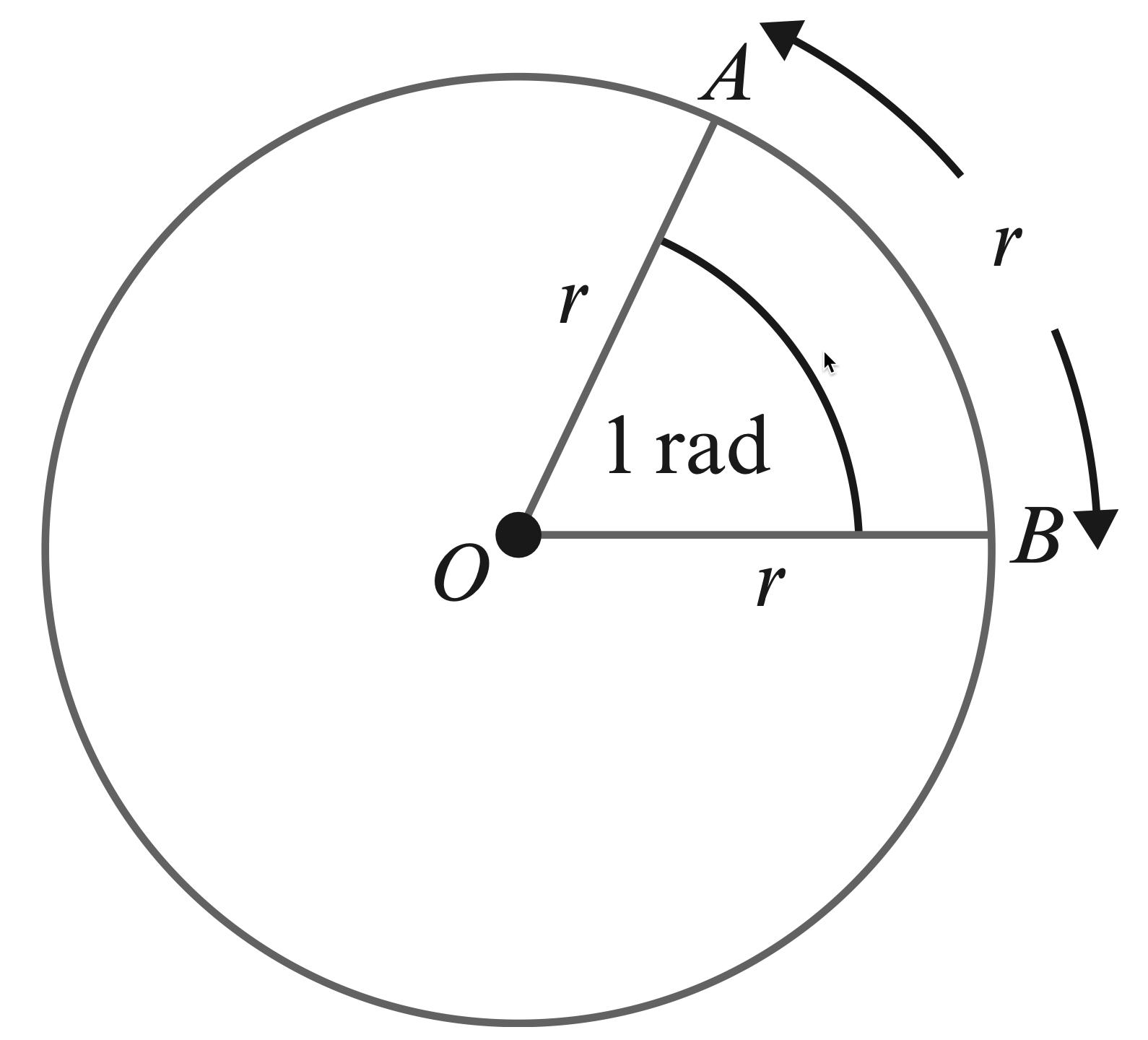An arc equal in length to the radius of a circle subtends an angle of $1$ radian at the centre.

Useful Relations

\begin{aligned} 2\pi \text{ radians}=360 \text{ degrees}\\\\ \pi \text{ radians}=180 \text{ degrees}\\\\ 1\text{ radian}=\dfrac{180}{\pi} \text{ degrees}\\\\ 1\text{ degree}=\dfrac{\pi}{180} \text{ radians}\\\\ \end{aligned}

Arc Length and Area of a Sector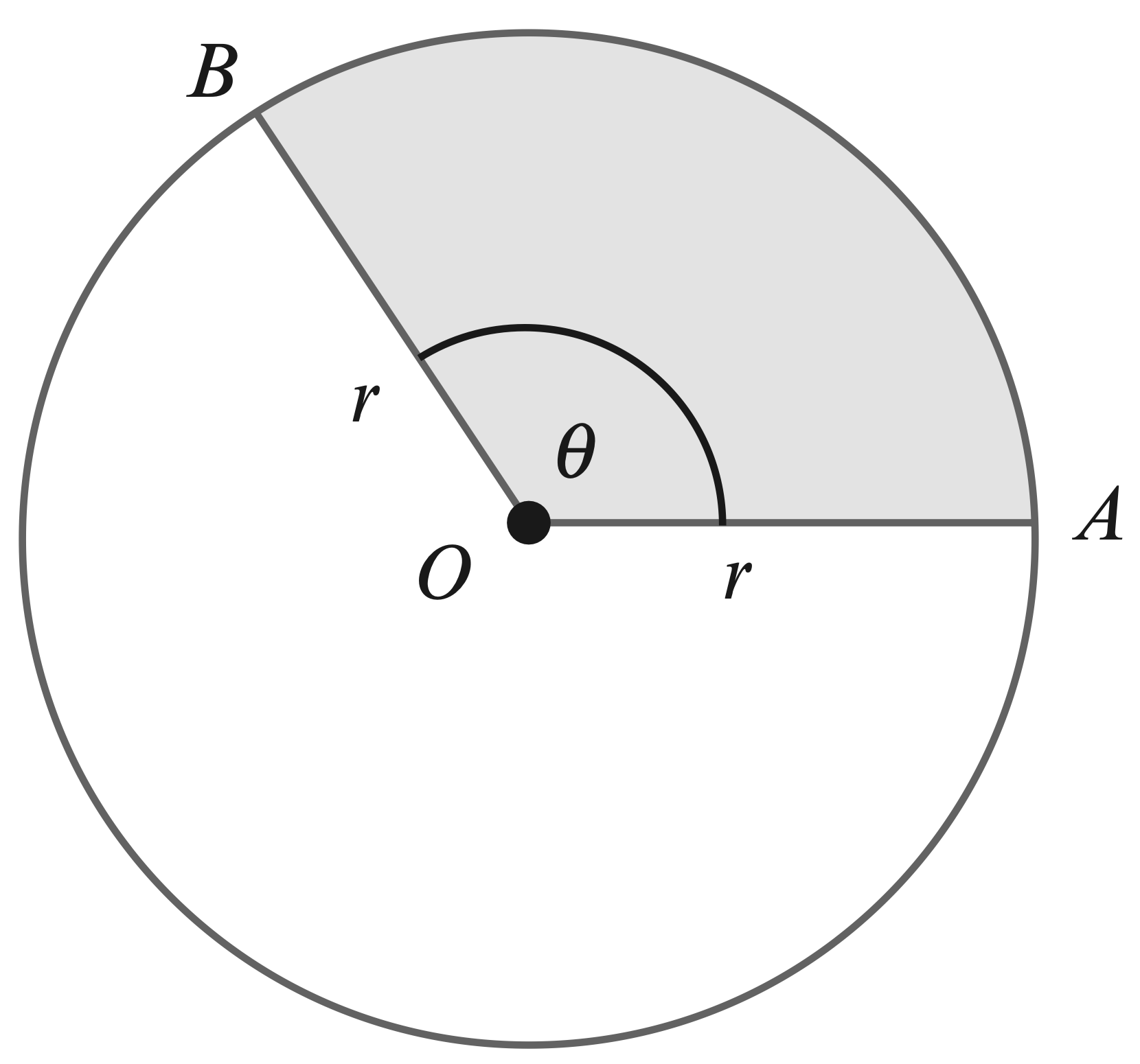• When $\theta$ is measured in radians, the length of arc $AB$ is $r\theta$ .

• When $\theta$ is measured in radians, the area of sector $AOB$ is $\dfrac{1}{2}r^2\theta$ .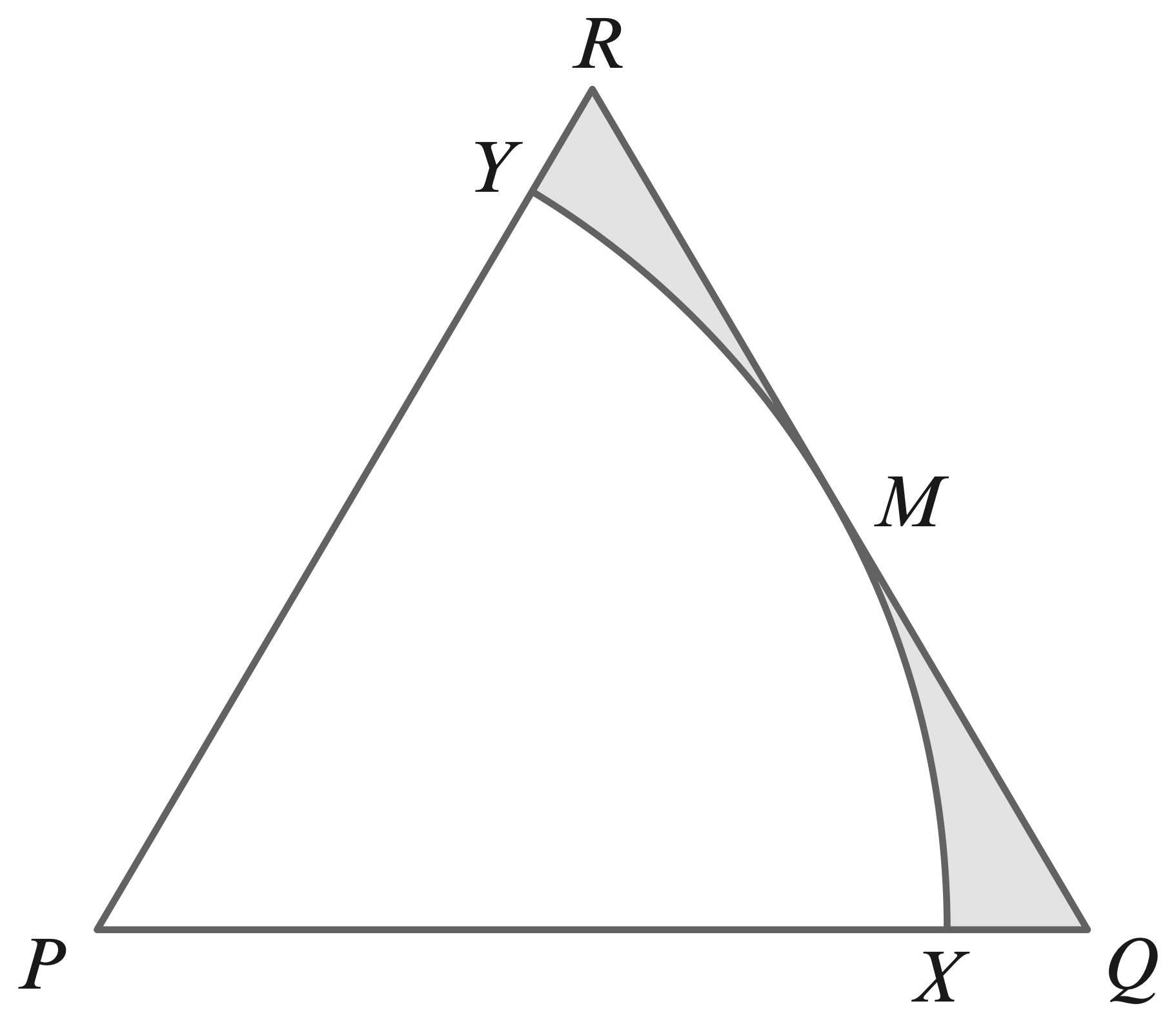1. The diagram shows ane quilateral triangle, $PQR$, with side length $5$ cm. $M$ is the midpoint of the line $QR$. An arc of a circle, centre $P$, touches $QR$ at $M$ and meets $PQ$ at $X$ and $PR$ at $Y$. Find in terms of $\pi$ and $\sqrt{3}:$
(a) the total perimeter of the shaded region.
(b) the total area of the shaded region.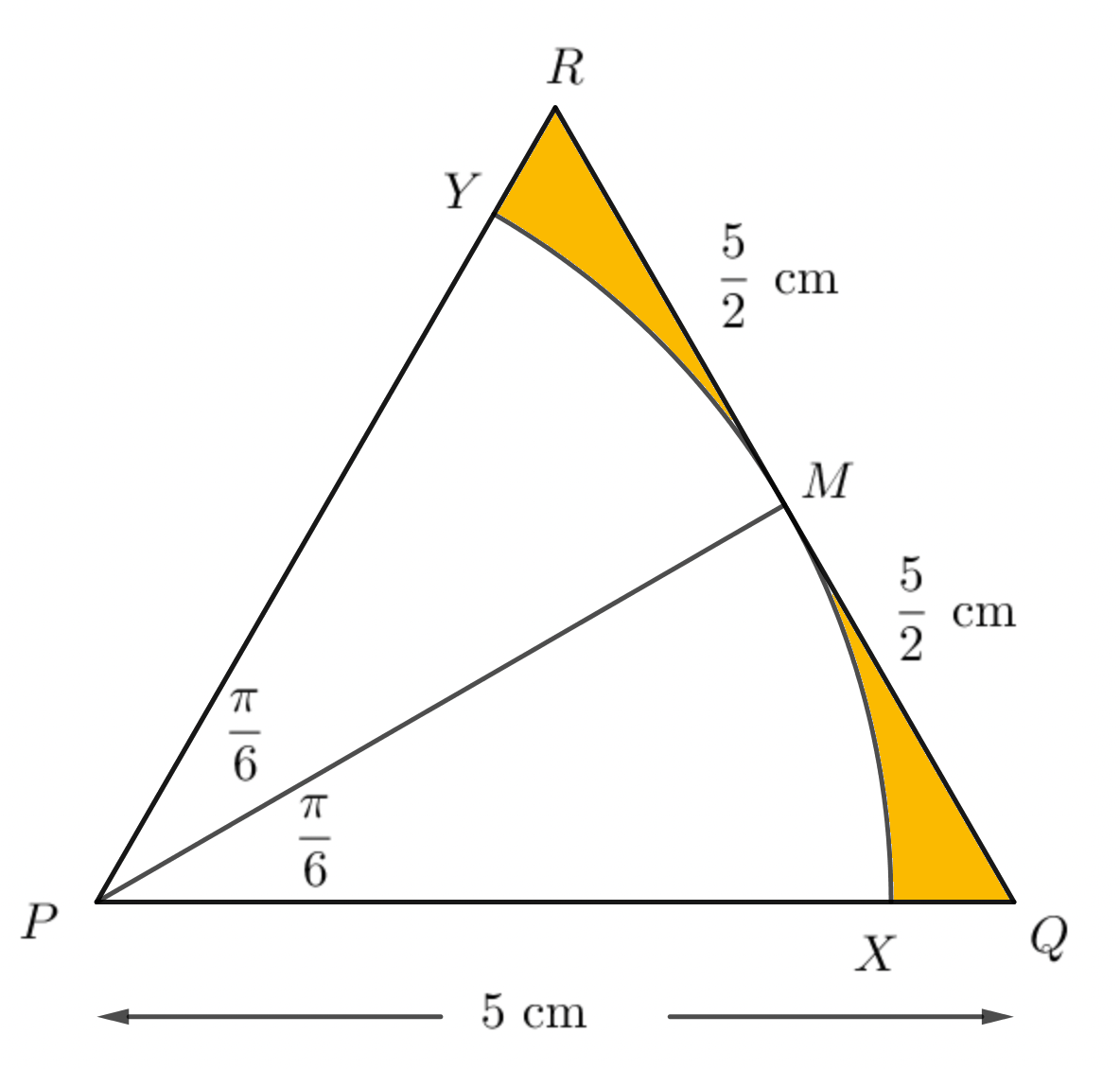Join PM, then $\triangle P R M$ and $\triangle P O M$ are $30^{\circ}-60^{\circ}$ right triangles,
\begin{aligned} &\\ \therefore \ P M &=\dfrac{\sqrt{3}}{2}\\\\ P Q&=\dfrac{5 \sqrt{3}}{2}\\\\ \text{arc length of } X Y &=P M \cdot \dfrac{\pi}{3}\\\\ &=\dfrac{5 \sqrt{3}}{2} \cdot \dfrac{\pi}{3}\\\\ &=\dfrac{5 \sqrt{3} \pi}{6} \mathrm{~cm}\\\\ \text{perimeter of shaded region} &=5+\dfrac{5 \sqrt{3} \pi}{6} \mathrm{~cm}\\\\ \text{total area of shaded region}& = \text{area of triangle - area of rector}&\\\\ &=\dfrac{\sqrt{3}}{4} \times 5^{2}-\dfrac{1}{2} \times \dfrac{5 \sqrt{3}}{2} \times \dfrac{\pi}{6} \\\\ &=\dfrac{25 \sqrt{3}}{4}-\dfrac{5 \sqrt{3} \pi}{24} \\\\ &=\dfrac{5 \sqrt{3}}{24}[30-\pi] \mathrm{cm}^{2} \end{aligned}

2.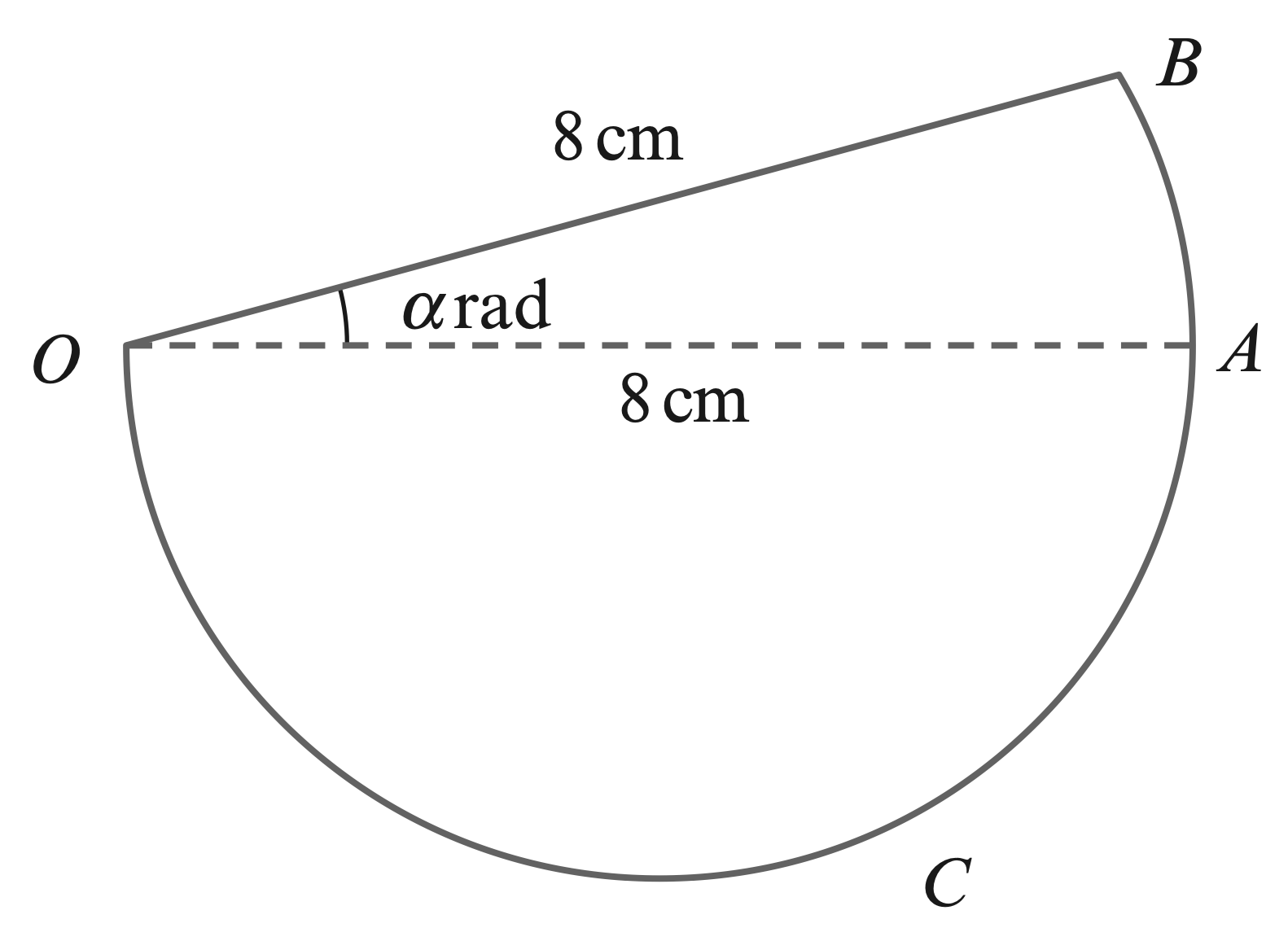3. In the diagram, $OAB$ is a sector of a circle with centre $O$ and radius $8$ cm. Angle $BOA$ is $\alpha$ radians. $OAC$ is a semicircle with diameter $OA$. The area of the semicircle $OAC$ is twice the area of the sector $OAB$.
(a) Find $\alpha$ in terms of $\pi$.
(b) Find the perimeter of the complete figure in terms of $\pi$.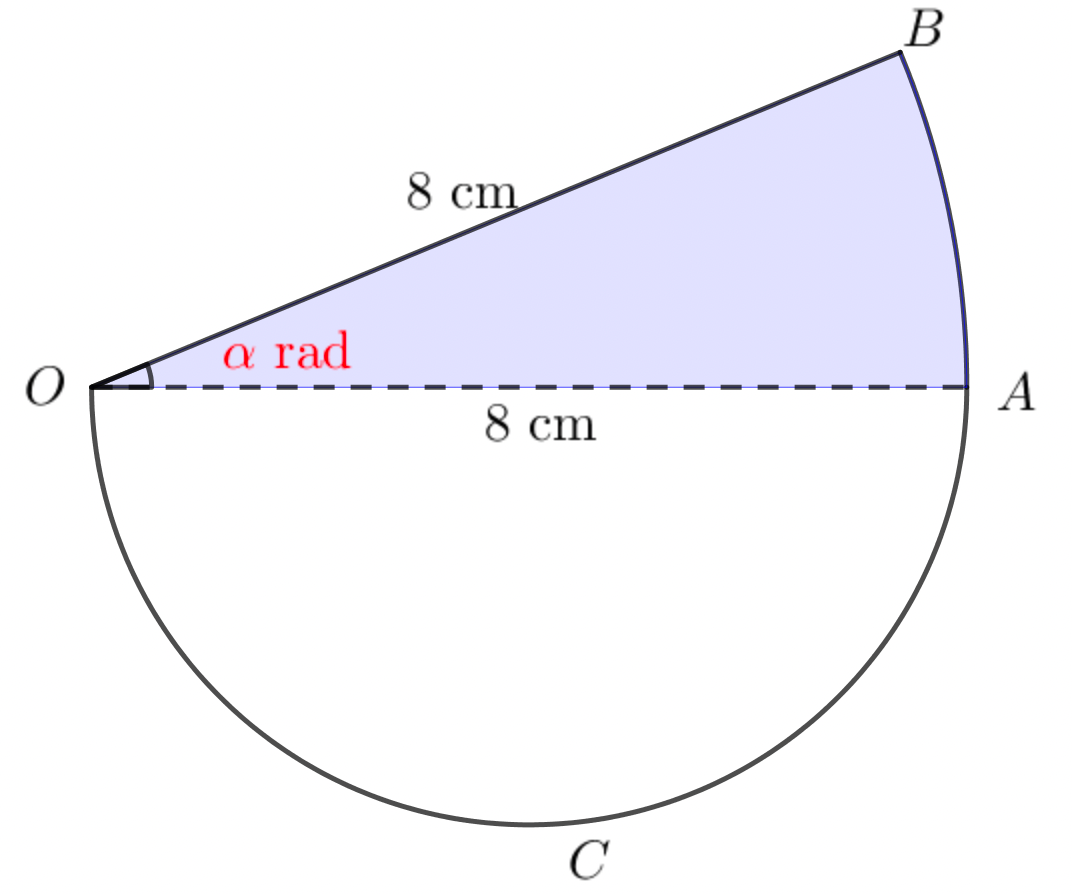\begin{aligned} \text{radius of sector } O A B &=8 \mathrm{~cm}\\\\ \text{radius of semicircle } O A C &=4 \mathrm{~cm}\\\\ \text{area of sector } O A B &=\dfrac{1}{2}\left(8^{2}\right) \alpha\\\\ &=32 \alpha\\\\ \text{area of semicircle } O A C &=\dfrac{1}{2}\left(4^{2}\right) \pi\\\\ &=8 \pi \mathrm{cm}^{2}\\\\ \text{By the problem},&\\\\ 8 \pi &=2(32 \alpha)\\\\ \alpha &=\dfrac{\pi}{8}\\\\ \therefore\ \text{length of arc } A B=8 \alpha\\\\ &=8\left(\dfrac{\pi}{8}\right) \\\\ &=\pi \mathrm{cm}\\\\ \end{aligned}
\begin{aligned} &\text{perimeter } \text{of given figure}\\\\ &=O B+\text { length of arc } A B+\text { length of semicircle } \\\\ &=8+\pi+4 \pi \\\\ &=8+5 \pi \end{aligned}

4.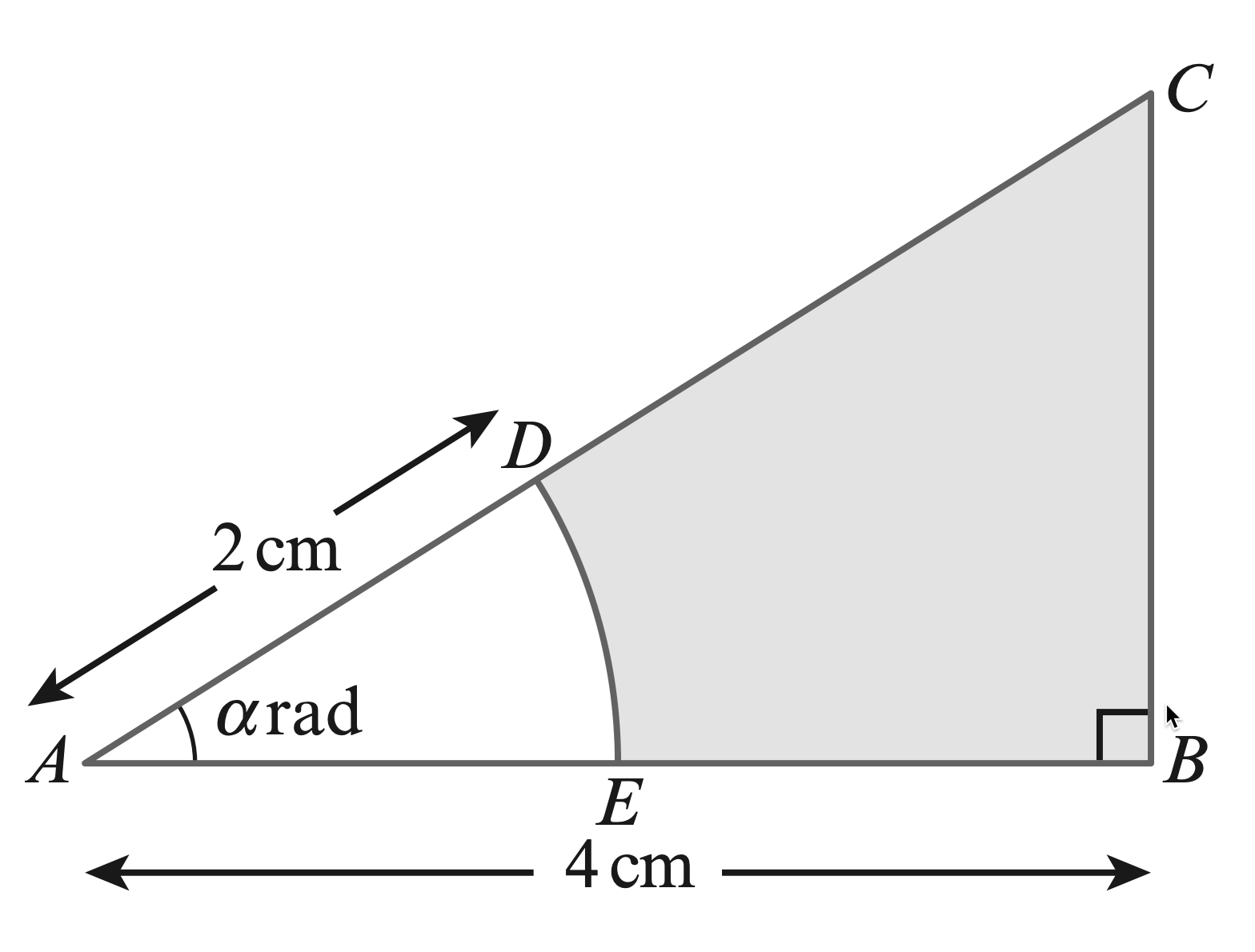5. The diagram shows triangle $ABC$ in which $AB$ is perpendicular to $BC$. The length of $AB$ is $4$ cm and angle $CAB$ is $\alpha$ radians. The arc $DE$ with centre $A$ and radius $2$ cm meets $AC$ at $D$ and $AB$ at $E$ . Find, in terms of $\alpha$,
(a) the area of the shaded region,
(b) the perimeter of the shaded region.

\begin{aligned} \text{length of arc } DE &=2\alpha \text{ cm }\\\\ BE &=2 \text{ cm }\\\\ \dfrac{BC}{AB} &=\ \tan\alpha\\\\ \therefore\ BC &=\ AB\tan\alpha\\\\ &=\ 4\tan\alpha \text{ cm }\\\\ \dfrac{AC}{AB} &=\ \sec\alpha\\\\ \therefore\ AC &=\ AB\sec\alpha\\\\ &=\ 4\sec\alpha \text{ cm }\\\\ \therefore\ DC &=\ 4\sec\alpha - 2\\\\ \end{aligned}
\begin{aligned} & \text{area of shaded region } \\\\ =&\ \text{area of triangle} - \text{ area of sector}\\\\ =&\ \dfrac{1}{2}(AB \cdot BC) - \dfrac{1}{2}AD^2\cdot\alpha\\\\ =&\ \dfrac{1}{2}(4 \cdot 4\tan\alpha) - \dfrac{1}{2}\cdot2^2\cdot\alpha\\\\ =&\ 8\tan\alpha - 2\cdot\alpha\\\\ =&\ 2(4\tan\alpha - \alpha)\\\\ & \text{perimeter of shaded region } \\\\ =&\ DC + BC + BE + \text{length of arc } DE\\\\ =&\ 4\sec\alpha - 2 + 4\tan\alpha + 2 + 2\alpha\\\\ =&\ 2(2\sec\alpha + 2\tan\alpha + \alpha) \end{aligned}

6.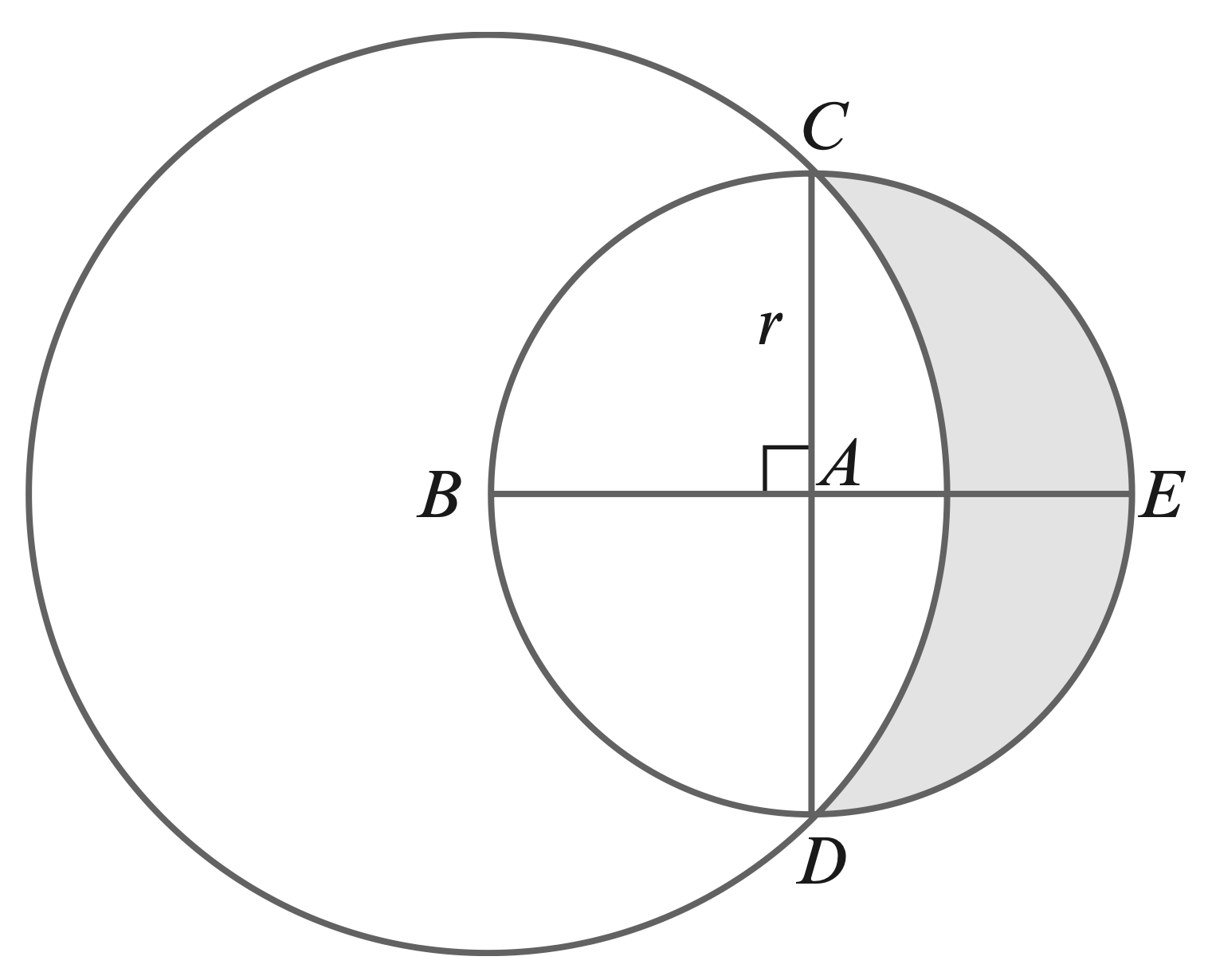7. The diagram shows a circle with centre $A$ and radius $r$. Diameters $CAD$ and $BAE$ are perpendicular to each other. A larger circle has centre $B$ and passes through $C$ and $D$.
(a) Show that the radius of the larger circle is $r\sqrt{2}$.
(b) Find the area of the shaded region in terms of $r$.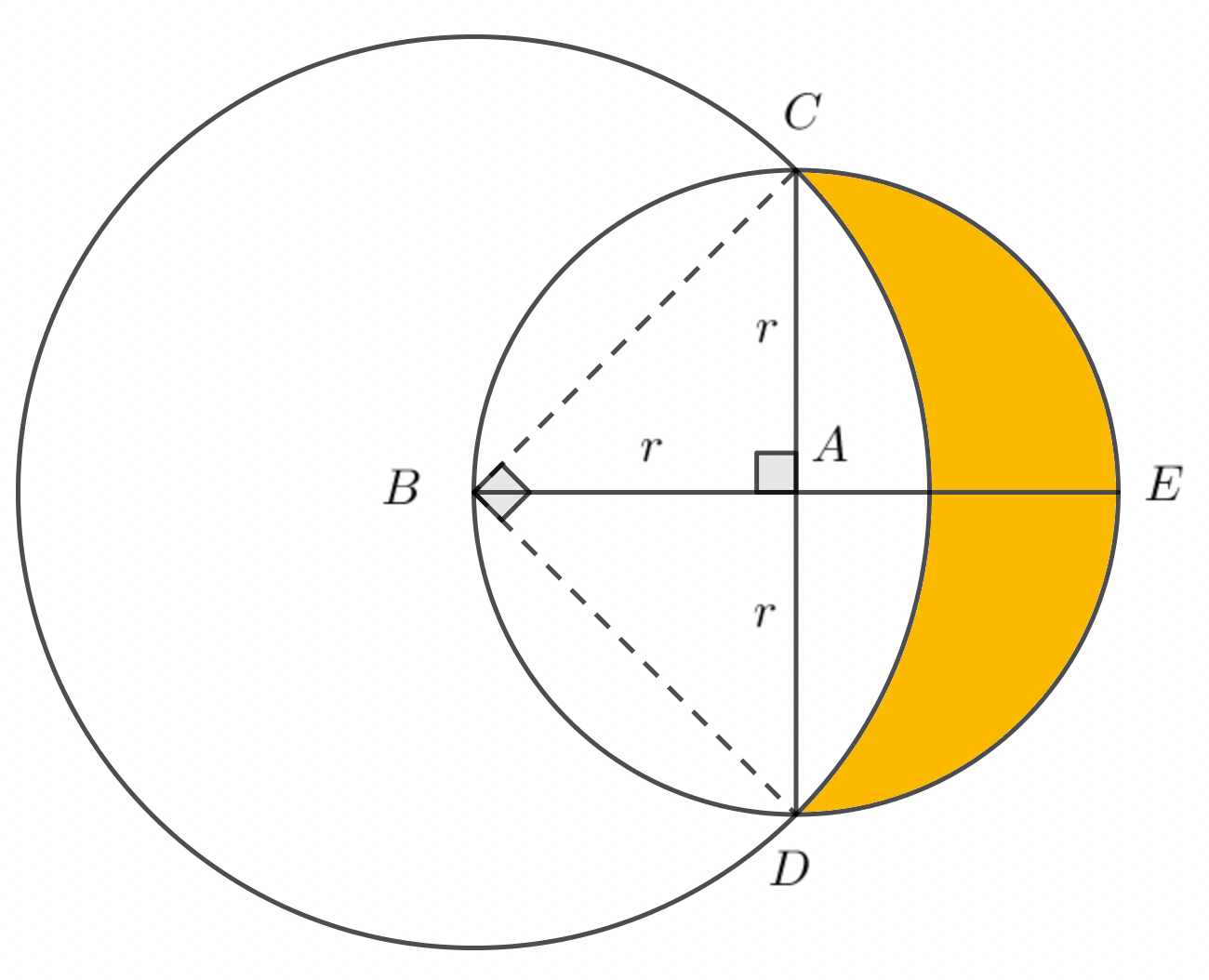$B C = \text{ radius of larger circle.}\\\\$
$\triangle B A C \text{is a } 45^{\circ}-45^{\circ} \text{ right triangle.}\\\\$
$\therefore\ B C=r \sqrt{2}$
\begin{aligned} & \\ A_{1}=\text { area of semicircle } C E D &=\dfrac{1}{2} \pi r^{2} \\\\ A_{2}=\text { area of sector } C B D &=\dfrac{1}{2} B C^{2} \times \angle C B D \\\\ &=\dfrac{1}{2}(r \sqrt{2})^{2} \times\left(\dfrac{\pi}{2}\right) \\\\ &=\dfrac{1}{2} \pi r^{2}\\\\ A_{3}=\text { area of } \triangle C B D &=\dfrac{1}{2} B C \times B D \\\\ &=\dfrac{1}{2}(r \sqrt{2})(r \sqrt{2}) \\\\ &=r^{2} \\\\ \therefore\ \text { area of shaded region } &=A_{1}-\left(A_{2}-A_{3}\right) \\\\ &=\dfrac{1}{2} \pi r^{2}-\left(\dfrac{1}{2} \pi r^{2}-r^{2}\right) \\\\ &= r^{2} \end{aligned}

8.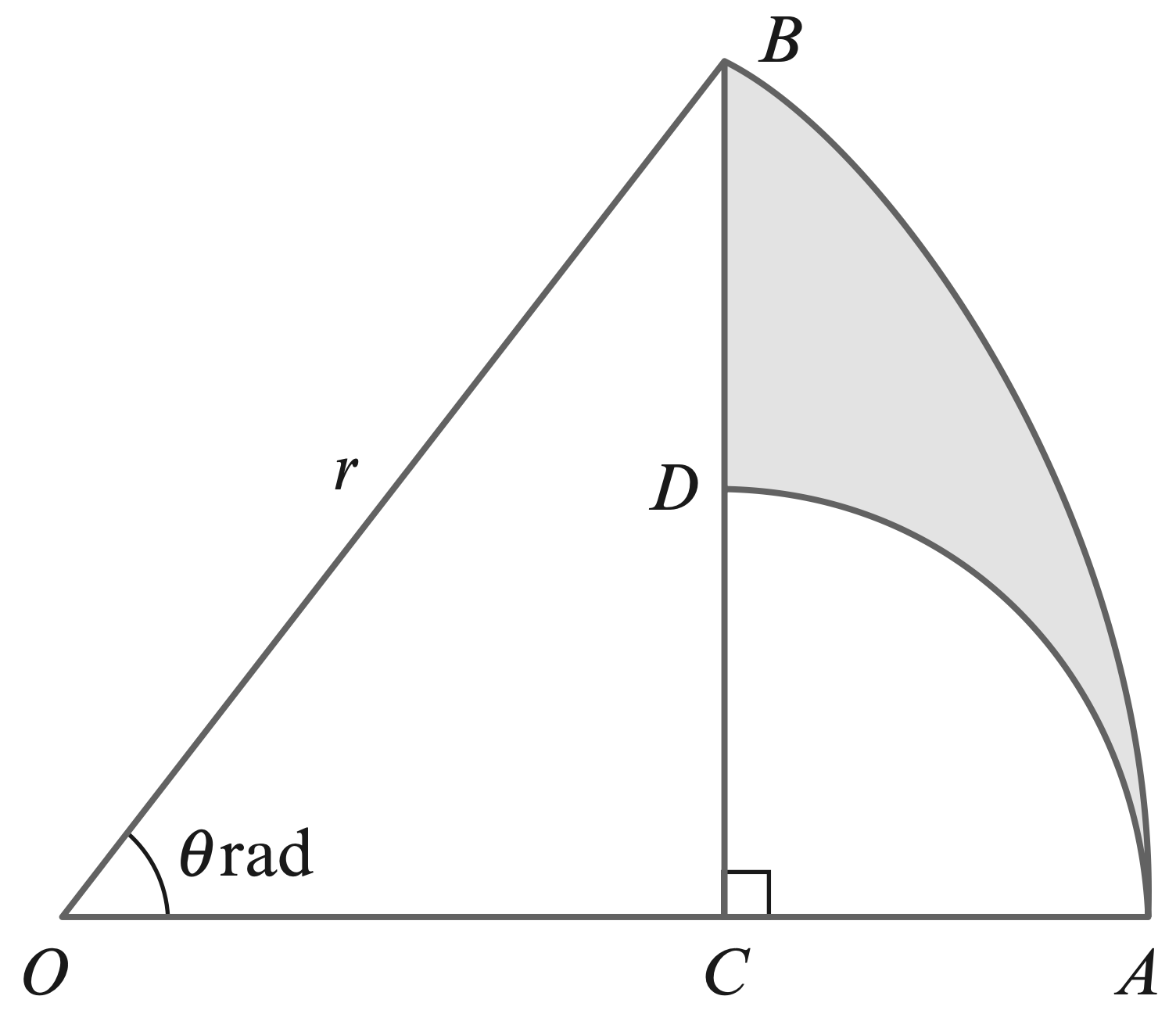9. The diagram shows a sector $OAB$ of a circle with centre $O$ and radius $r$. Angle AOB is $\theta$ radians. The point $C$ on $OA$ is such that $BC$ is perpendicular to $OA$. The point $D$ is on $BC$ and the circular arc $AD$ has centre $C$. (a) Find $AC$ in terms of $r$ and $\theta$. (b) Find the perimeter of the shaded region $ABD$ when $\theta= \dfrac{1}{3}\pi$ and $r = 4$, giving your answer as an exact value.

\begin{aligned} \triangle O B C & \text{ is a right triangle.}\\\\ \therefore B C &=r \sin \theta \\\\ O C &=r \cos \theta \\\\ A C &=O A-O C \\\\ &=r-r \cos \theta \\\\ &=r(1-\cos \theta) \\\\ C D &=A C\ \ (\because \text { radii of small sector }) \\\\ \therefore\ C D &=r(1-\cos \theta)\\\\ r=4, \theta &=\dfrac{\pi}{3} \text { (given) } \\\\ \textbf { length of arc } A D &=A C \times \dfrac{\pi}{2} \\\\ &=\dfrac{\pi r}{2}(1-\cos \theta) \\\\ &=\dfrac{\pi \times 4}{2}\left(1-\cos \dfrac{\pi}{3}\right) \\\\ &=\pi \\\\ B D &=B C-C D \\\\ &=r \sin \theta-r(1-\cos \theta) \\\\ &=r(\sin \theta+\cos \theta-1)\\\\ &=4\left(\sin \dfrac{\pi}{3}+\cos \dfrac{\pi}{3}-1\right) \\\\ &=4\left(\dfrac{\sqrt{3}}{2}+\dfrac{1}{2}-1\right) \\\\ &=2(\sqrt{3}-1) \\\\ \textbf { length of arc } A B &=r \theta \\\\ &=\dfrac{4 \pi}{3} \\\\ \therefore\ \textbf{ Perimeter of shaded} & \textbf{ region }\\\\ &=\pi+\dfrac{4 \pi}{3}+2(\sqrt{3}-1) \\\\ &=\dfrac{7 \pi}{3}+2(\sqrt{3}-1) \end{aligned}

10.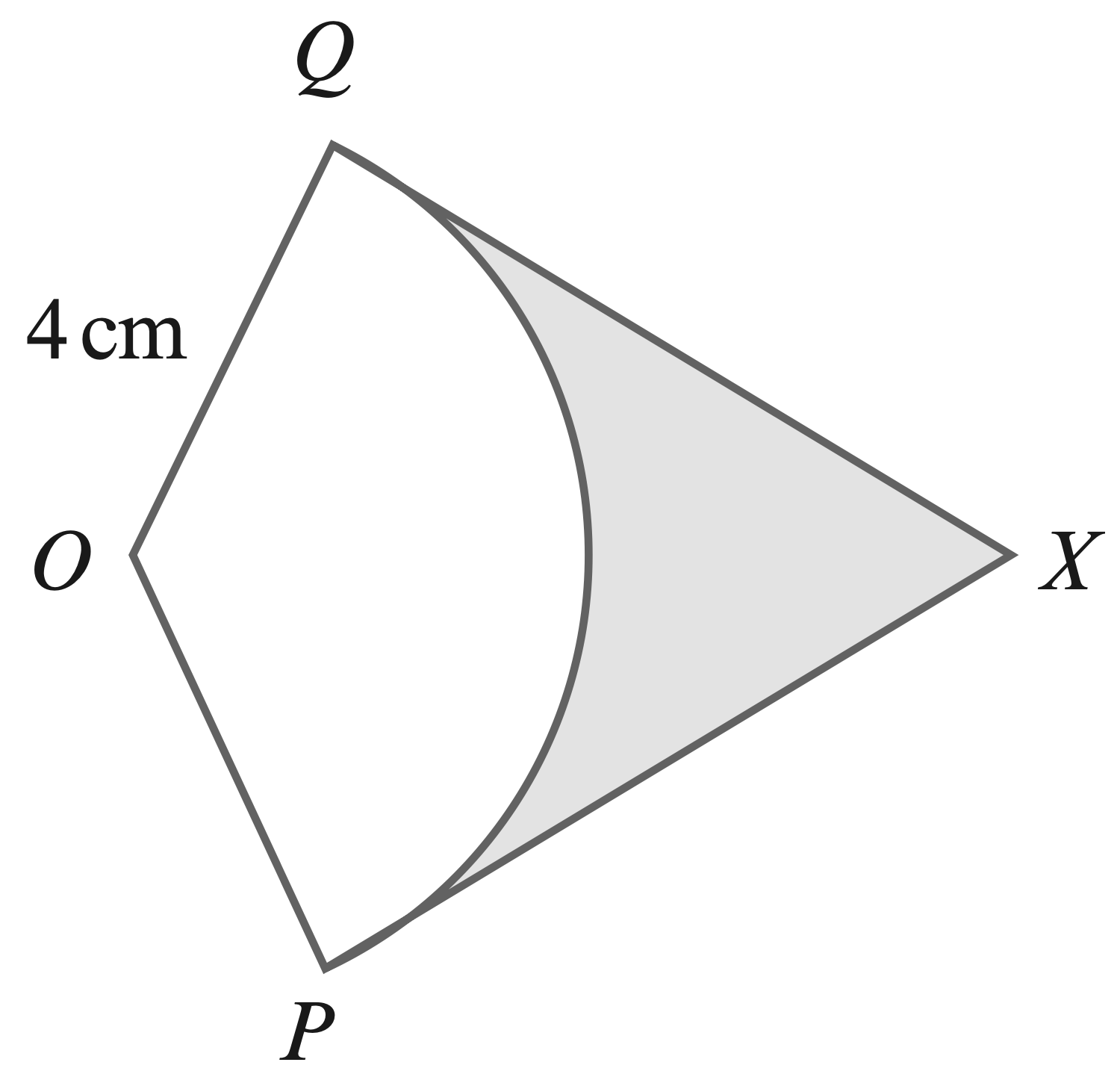11. The diagram shows a sector, $P O Q$, of a circle, centre $O$, with radius $4 \mathrm{~cm}$. The length of arc $P Q$ is $7 \mathrm{~cm}$. The lines $P X$ and $Q X$ are tangents to the circle at $P$ and $Q$, respectively.
(a) Find angle $P O Q$, in radians.
(b) Find the length of $P X$.
(c) Find the area of the shaded region.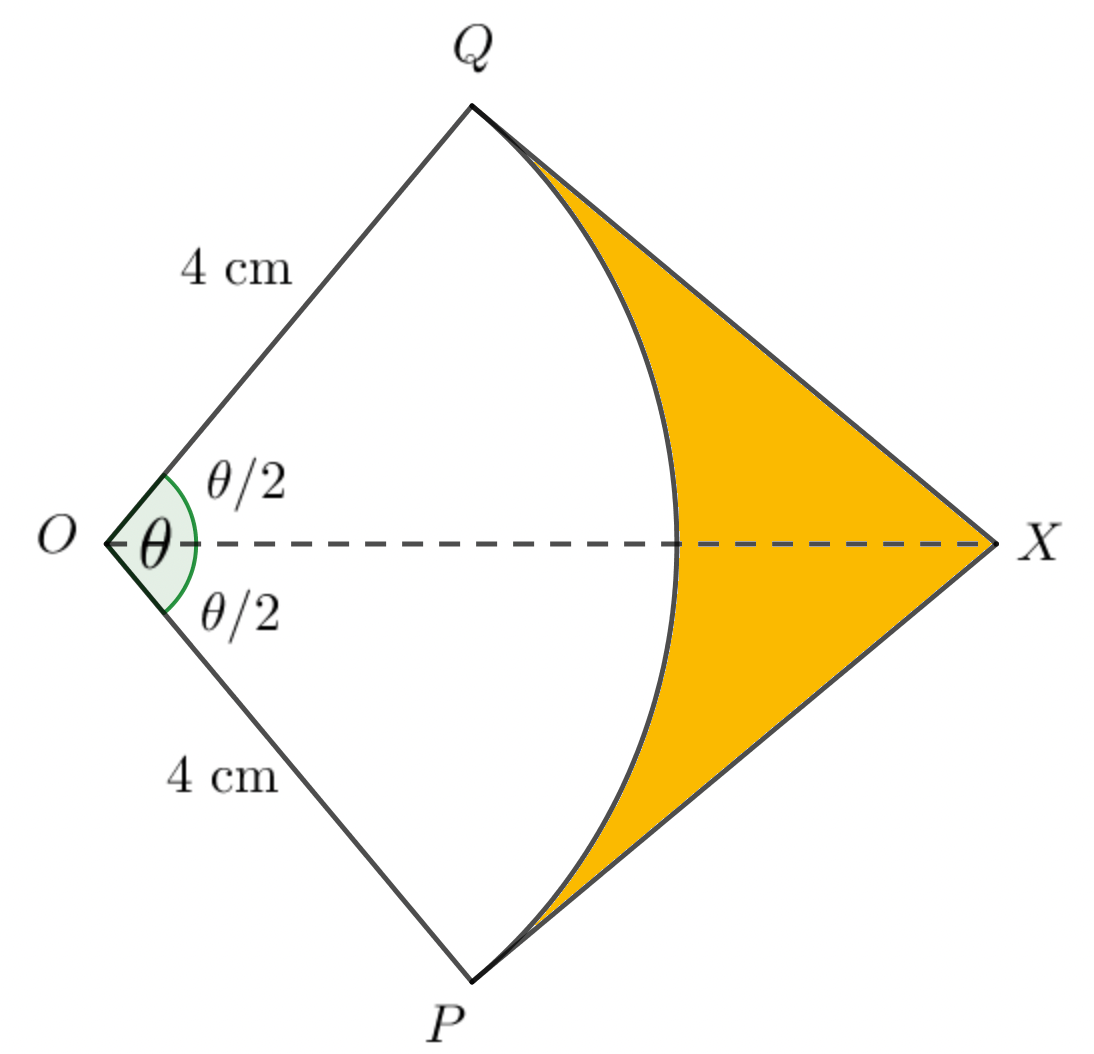radius $(r)=4 \mathrm{~cm}\\\\$
length of arc $P Q(s)=7 \mathrm{~cm}\\\\$
$\angle P O Q(\theta)=\dfrac{3}{8}=\dfrac{7}{4} \text{ radians}\\\\$
$\triangle P O X \text{ is a right triangle.}\\\\$
\begin{aligned} \therefore P X &=O P \tan \left(\dfrac{\theta}{2}\right) \\\\\ &=4 \tan \left(\dfrac{7}{8}\right) \mathrm{cm}\\\\ \end{aligned}
\begin{aligned} &\text{area of shaded region}\\\\ =&\ 2 \times \text { area of } \Delta P O \times-\text { area of sector POQ } \\\\ =&\ 2\left(\dfrac{1}{2} \times O P \times P X\right)-\dfrac{1}{2} O P^{2} \times \theta \\\\ =&1\ 6 \tan \left(\dfrac{7}{8}\right)-\dfrac{1}{2} \times 16 \times \dfrac{7}{4} \\\\ =&\ 16 \tan \left(\dfrac{7}{8}\right)-14 \mathrm{~cm}^{2} \end{aligned}

12.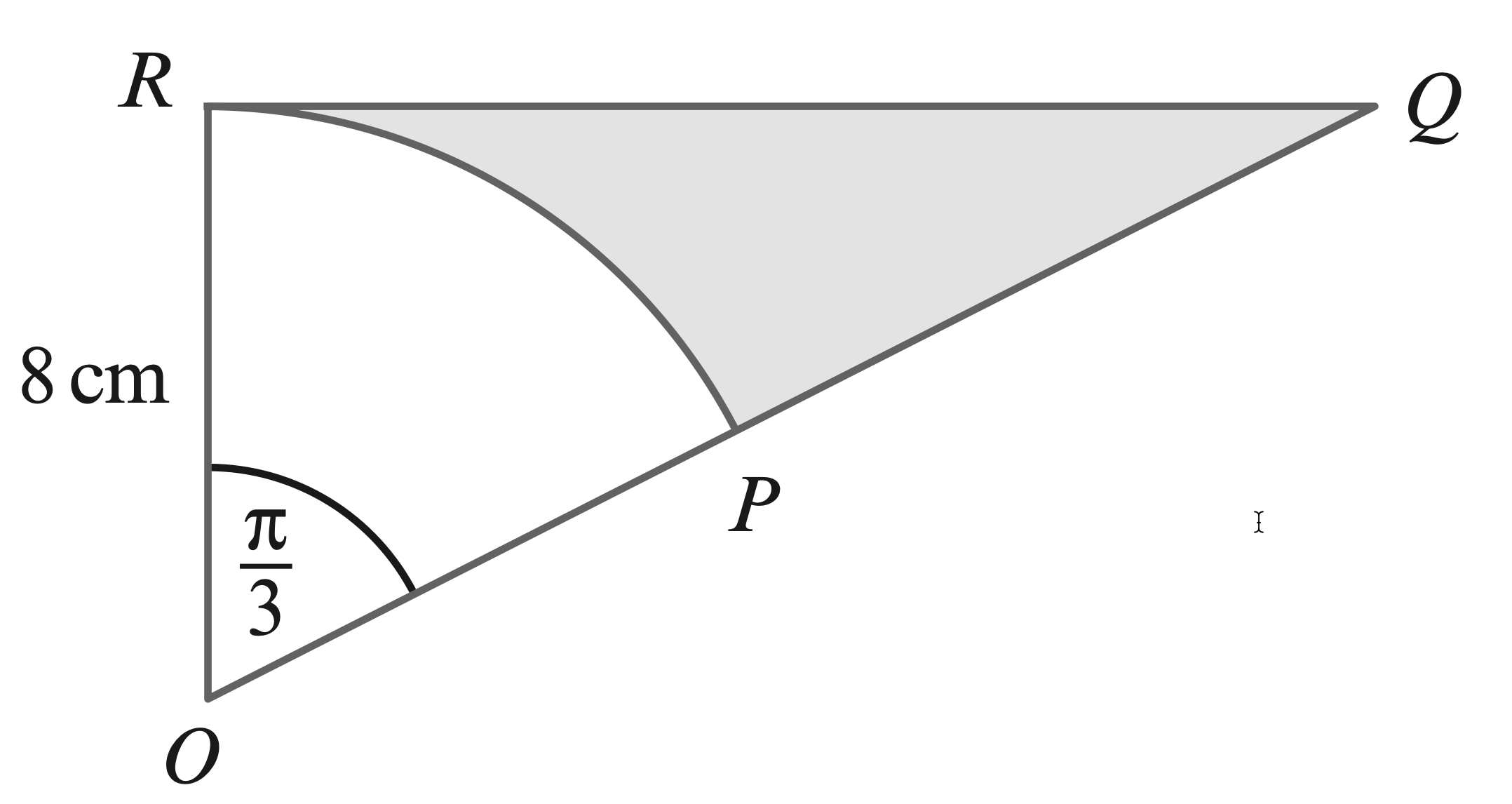13. The diagram shows a sector, $P O R$, of a circle, centre $O$, with radius $8 \mathrm{~cm}$ and sector angle $\dfrac{\pi}{3}$ radians. The lines $O R$ and $Q R$ are perpendicular and $O P Q$ is a straight line. Find the exact area of the shaded region.

$\triangle O Q R \text{ is a } 30^{\circ}-60^{\circ}\text{ right triangle.}\\\\$
$\therefore\ QR=8 \sqrt{3}\\\\$
\begin{aligned} \text{area of }\triangle OQR &=\dfrac{1}{2} \times 8 \times 8 \sqrt{3} \\\\ &=32 \sqrt{3} \mathrm{~cm}^{2} \\\\ \text{area of sector } OPR &=\dfrac{1}{2} \times 8^{2} \times \dfrac{\pi}{3}\\\\ &=\dfrac{32 \pi}{3} \mathrm{~cm}^{2}\\\\ \end{aligned}
\begin{aligned} & \text{ area of shaded region}\\\\ & \text{area of } \triangle O Q R - \text{area of sector } OPR\\\\ =&\ 32 \sqrt{3}-\dfrac{32 \pi}{3} \\ =&\ \dfrac{32}{3}(3 \sqrt{3}-\pi) \mathrm{cm}^{2} \end{aligned}

14.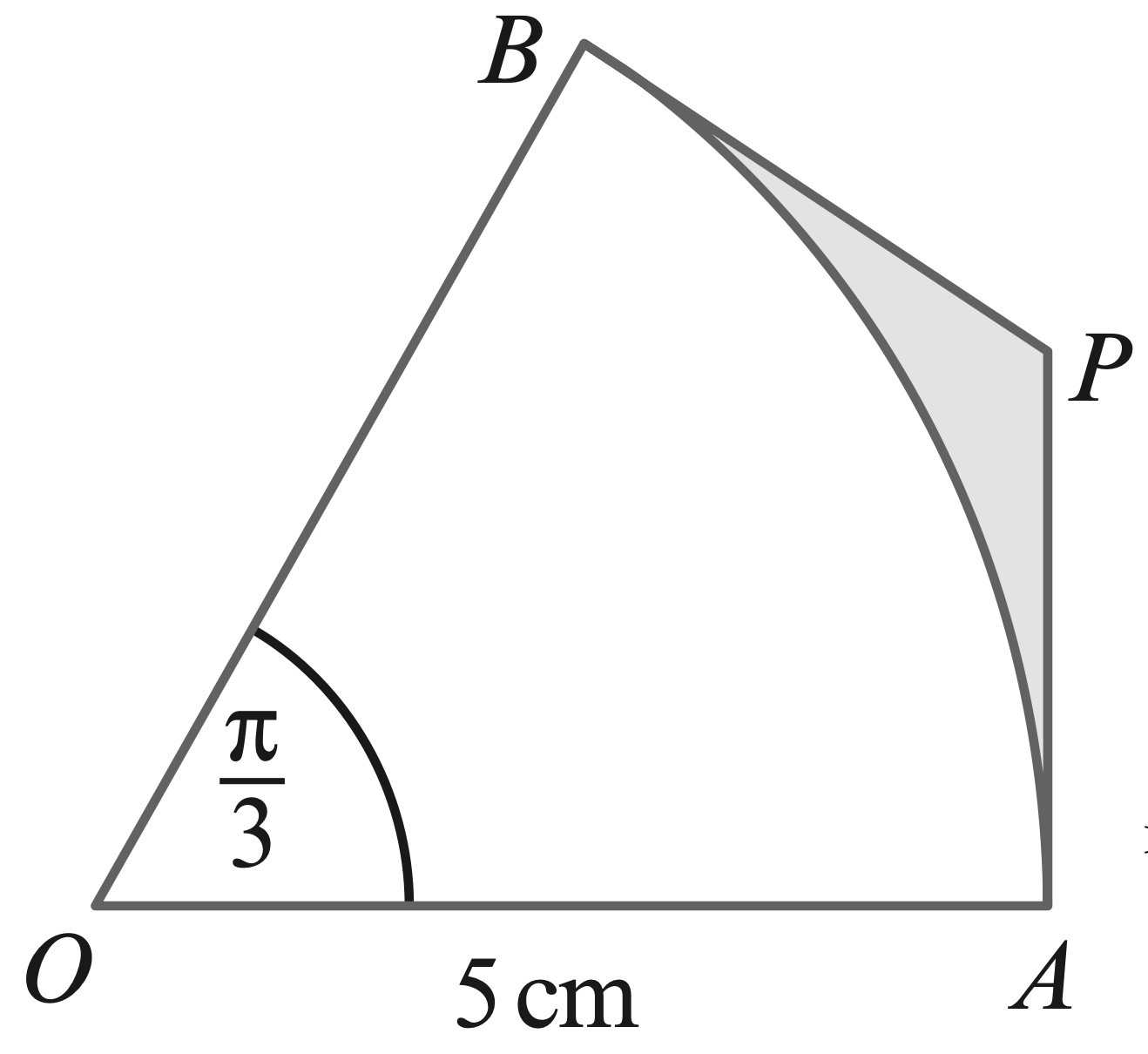15. The diagram shows a sector, $A O B$, of a circle, centre $O$, with radius $5 \mathrm{~cm}$ and sector angle $\dfrac{\pi}{3}$ radians. The lines $A P$ and $B P$ are tangents to the circle at $A$ and $B$, respectively.
(a) Find the exact length of $A P$.
(b) Find the exact area of the shaded region.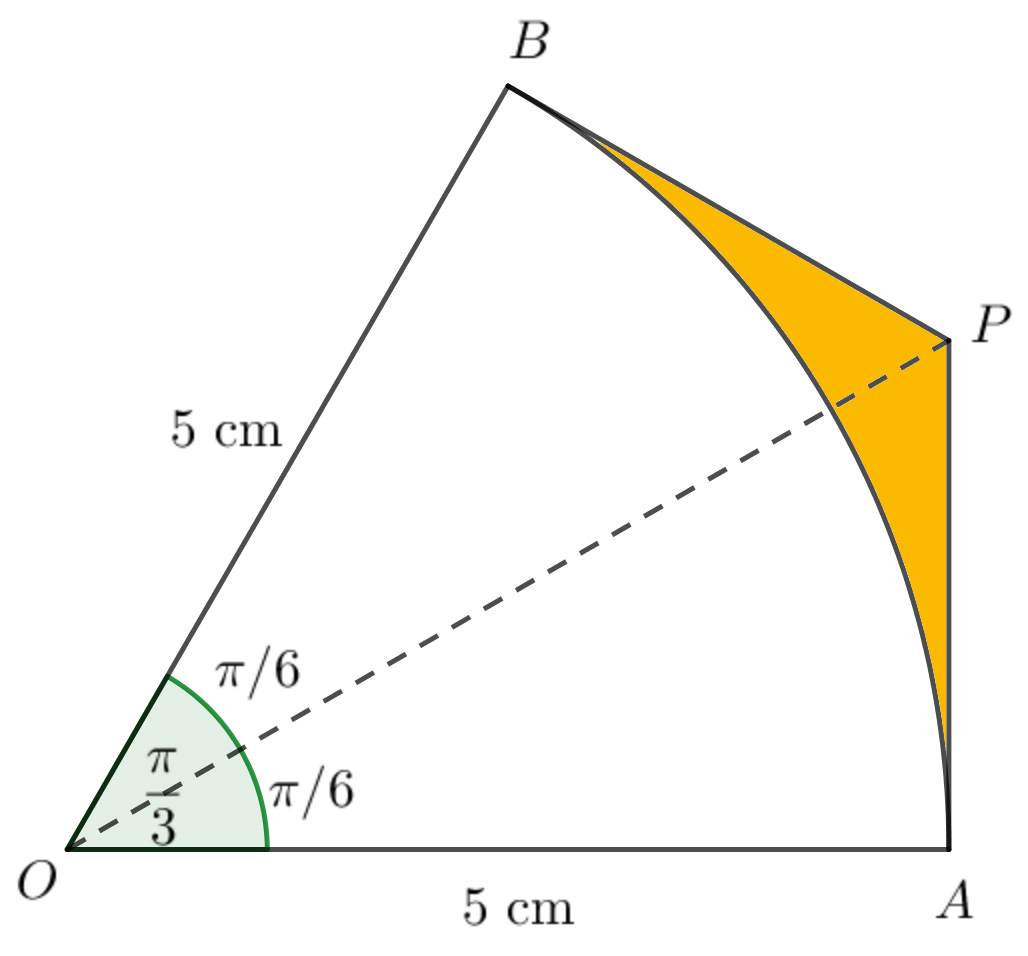$\text{Since } AP \text{ is a tangent,}\\\\$
$\triangle O A P \text{ is a right triangle.}\\\\$
$\therefore\ A P=5 \tan \left(\dfrac{\pi}{6}\right)\\\\$
$\quad \text{area of shaded region}\\\\$
$=\ 2 \times$ area of $\triangle O A P$ - area of sector $A O B\\\\$
$=\ 2\left(\dfrac{1}{2} \times O A \times A P\right)-\dfrac{1}{2} O A^{2} \times \angle A O B\\\\$
$=\ 25 \tan \left(\dfrac{\pi}{6}\right)-\dfrac{25}{2} \times \dfrac{\pi}{3}\\\\$
$=\ 25 \tan \left(\dfrac{\pi}{6}\right)-\dfrac{25 \pi}{6}\\\\$
$=\ 25\left[\tan \left(\dfrac{\pi}{6}\right)-\dfrac{\pi}{6}\right]$

16.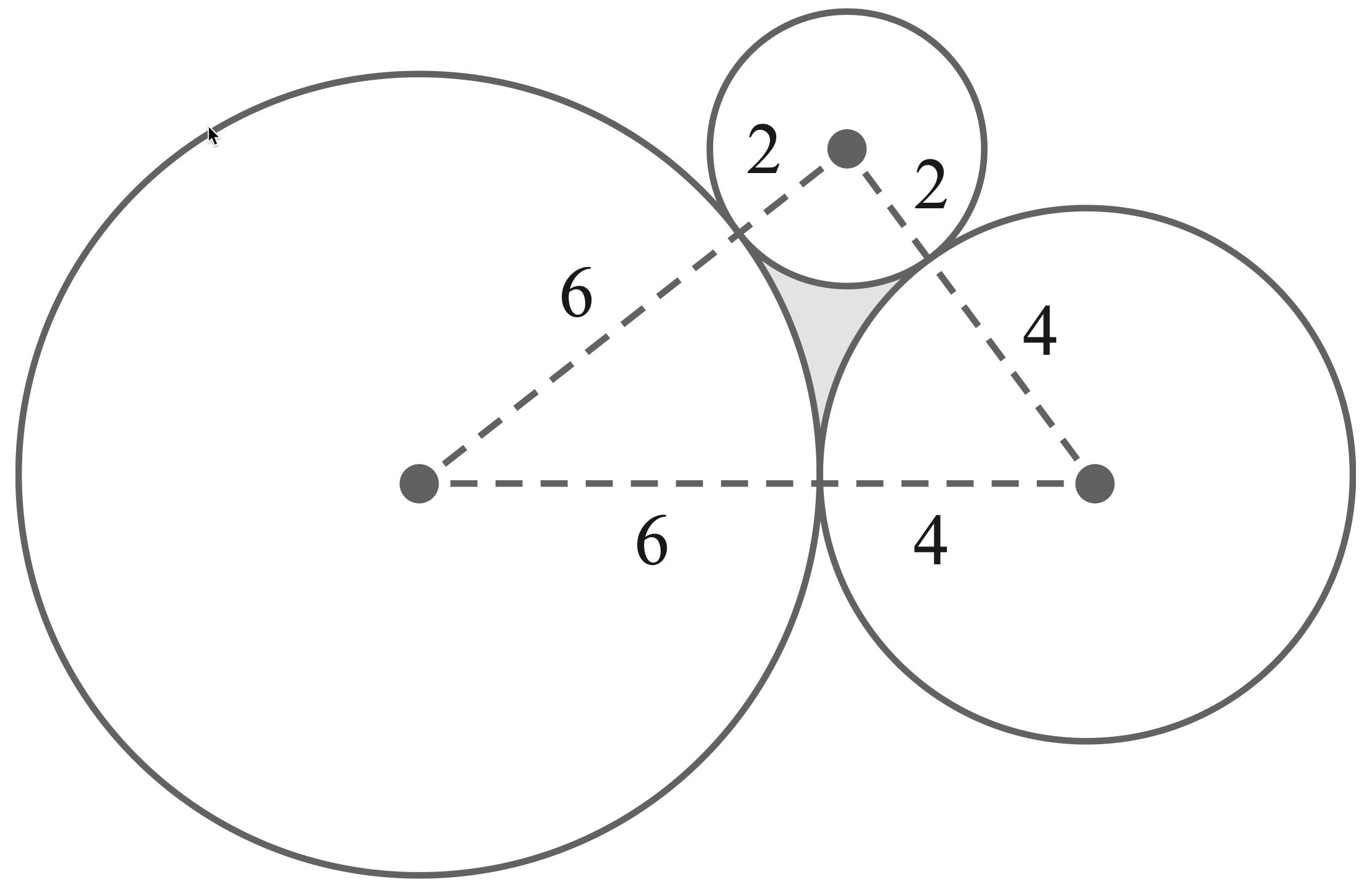17. The diagram shows three touching circles with radii $6 \mathrm{~cm}, 4 \mathrm{~cm}$ and $2 \mathrm{~cm}$. Find the area of the shaded region.

Since $A C^{2}+B C^{2}=8^{2}+6^{2}=100$ and $A B^{2}=10^{2}=100.\\\\$
$A B^{2}=A C^{2}+B C^{2}.\\\\$
$\therefore \triangle A B C$ is a right triangle.
\begin{aligned} &\\ \angle A&=\tan ^{-1}\left(\dfrac{3}{4}\right) \\\\ \angle B&=\tan ^{-1}\left(\dfrac{4}{3}\right) \\\\ \angle C&=\dfrac{\pi}{2}\\\\ \text { area of } \triangle A B C &=\dfrac{1}{2} \times A C \times B C \\\\ &=\dfrac{1}{2} \times 8 \times 6 \\\\ &=24 \mathrm{~cm}^{2} \\\\ P=\dfrac{1}{2} \times 6^{2} \times \tan ^{-1}\left(\dfrac{3}{4}\right) &=18 \tan ^{-1}\left(\dfrac{3}{4}\right) \mathrm{cm}^{2} \\\\ g=\dfrac{1}{2} \times 2^{2} \times \dfrac{\pi}{2} &=\pi \mathrm{cm}^{2} \\\\ R=\dfrac{1}{2} \times 4^{2} \times \tan ^{-1}\left(\dfrac{4}{3}\right) &=8 \tan ^{-1}\left(\dfrac{4}{3}\right) \mathrm{cm}^{2}\\\\ \end{aligned}
\begin{aligned} &\text{area of shaded region}\\\\ =& \ \text { area of } \triangle A B C-(P+8+R) \\ =&\ 24-\left(18 \tan ^{-1}\left(\dfrac{3}{4}\right)+\pi+8 \tan ^{-1}\left(\dfrac{4}{3}\right)\right) \\ =&\ 1.857 \mathrm{~cm}^{2} \end{aligned}

18.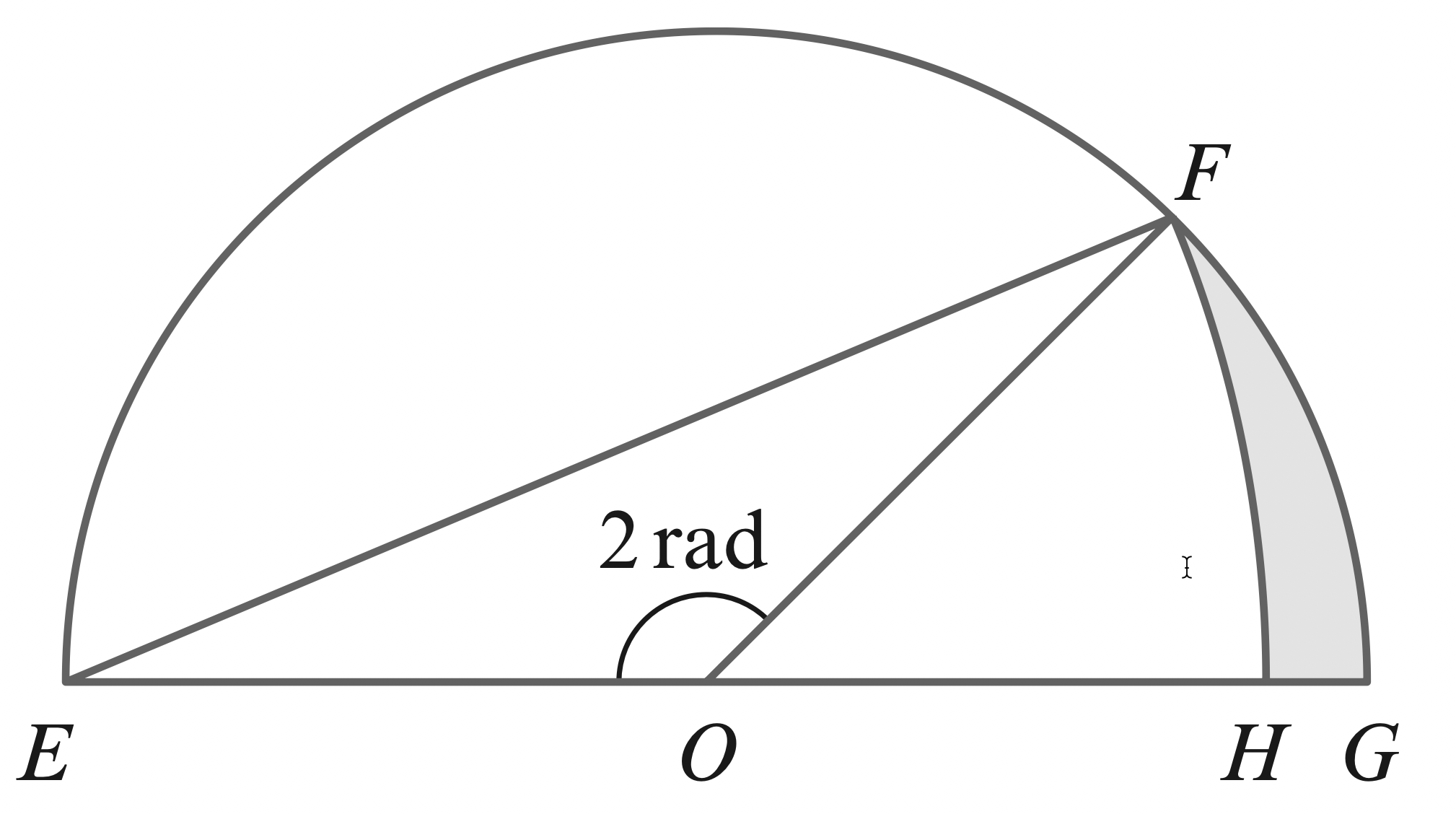19. The diagram shows a semicircle, centre $O$, with radius $8 \mathrm{~cm} .$ $F H$ is the arc of a circle, centre $E$. Find the area of:
(a) triangle $E O F$
(b) sector $F O G$
(c) sector $F E H$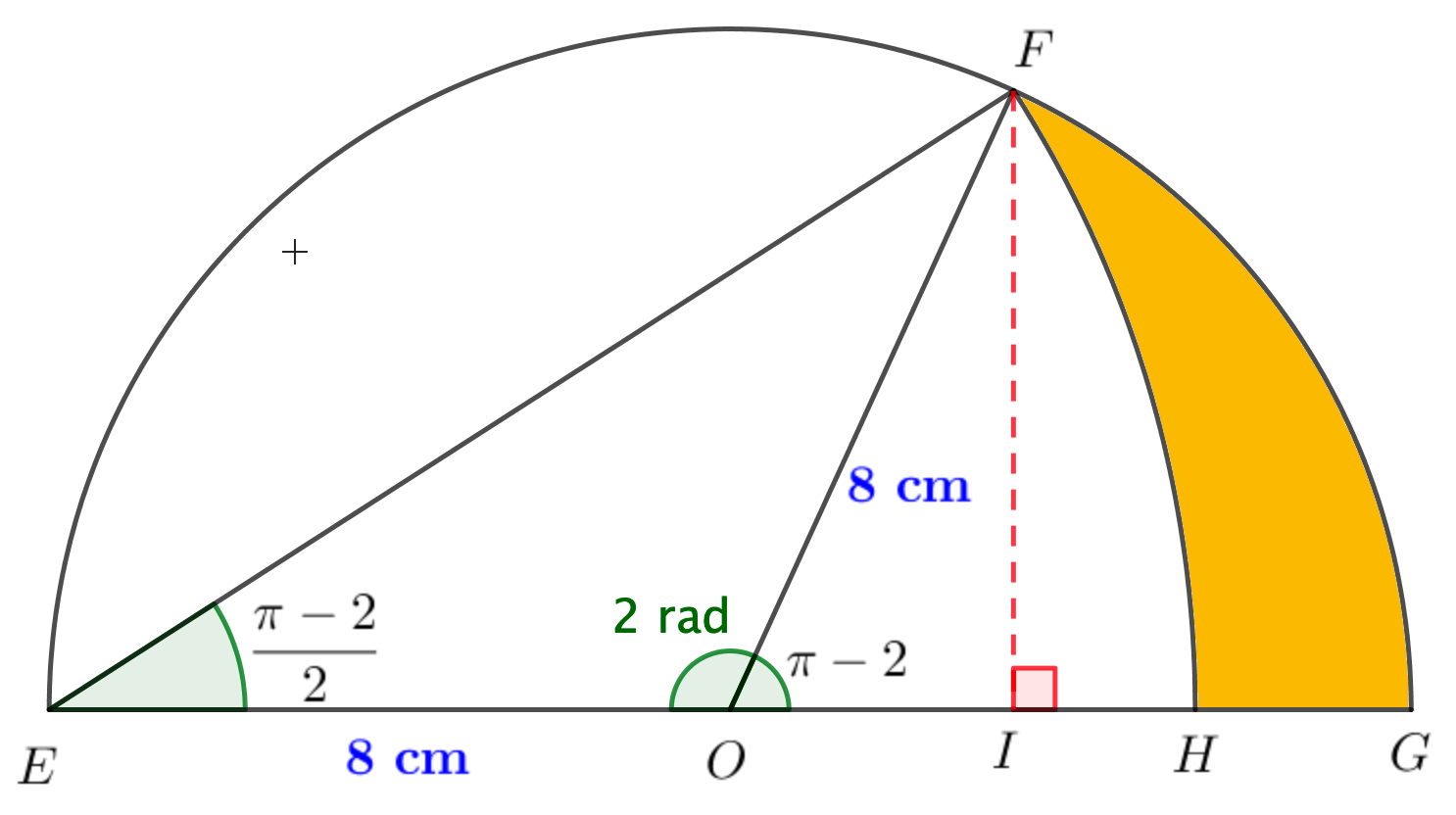\begin{aligned} &\textbf{area of } \triangle E O F\\\\ =&\ \dfrac{1}{2} \times E O \times F I \\\\ =&\ \dfrac{1}{2} \times 8 \times 8 \sin (\pi-2) \\\\ =&\ 32 \sin (\pi-2) \mathrm{cm}^{2}\\\\ &\textbf{area of sector } FOG\\\\ =&\ \dfrac{1}{2} \times O F^{2} \times \angle F O G \\\\ =&\ \dfrac{1}{2} \times 8^{2} \times(\pi-2) \\\\ =&\ 32(\pi-2) \mathrm{cm}^{2} \\\\ \angle F E H =&\ \dfrac{1}{2} \angle F O G \\\\ =&\ \dfrac{1}{2}(\pi-2)\\\\ E F^{2}=& 8^{2}+8^{2}-2\left(8^{2}\right) \cos (2) \\\\ =& 128(1-\cos (2)) \\\\ & \textbf{ area of sector FEH } \\\\ =&\ \dfrac{1}{2} E F^{2} \times \angle F E H \\\\ =&\ \dfrac{1}{2} \times 128 \times(1-\cos (2)) \times \dfrac{1}{2}(\pi-2) \\\\ =&\ 32(\pi-2)(1-\cos (2)) \mathrm{cm}^{2}\\\\ & \ \textbf{area of shaded region}\\\\ =&\ \textbf{area of sector } FOG- \textbf{area of } FOH\\\\ =&\ \textbf{ area of sector } FOG- (\textbf{area of sector } FEH - \textbf{area of } \triangle EOF) \\\\ =&\ 32(\pi-2)-32(\pi-2)(1-\cos (2))+32 \sin (\pi-2) \\\\ =&\ 32[(\pi-2) \cos (2)+\sin (\pi-2)] \\\\ =&\ 13.895 \mathrm{~cm}^{2}\\\\ \end{aligned}

20.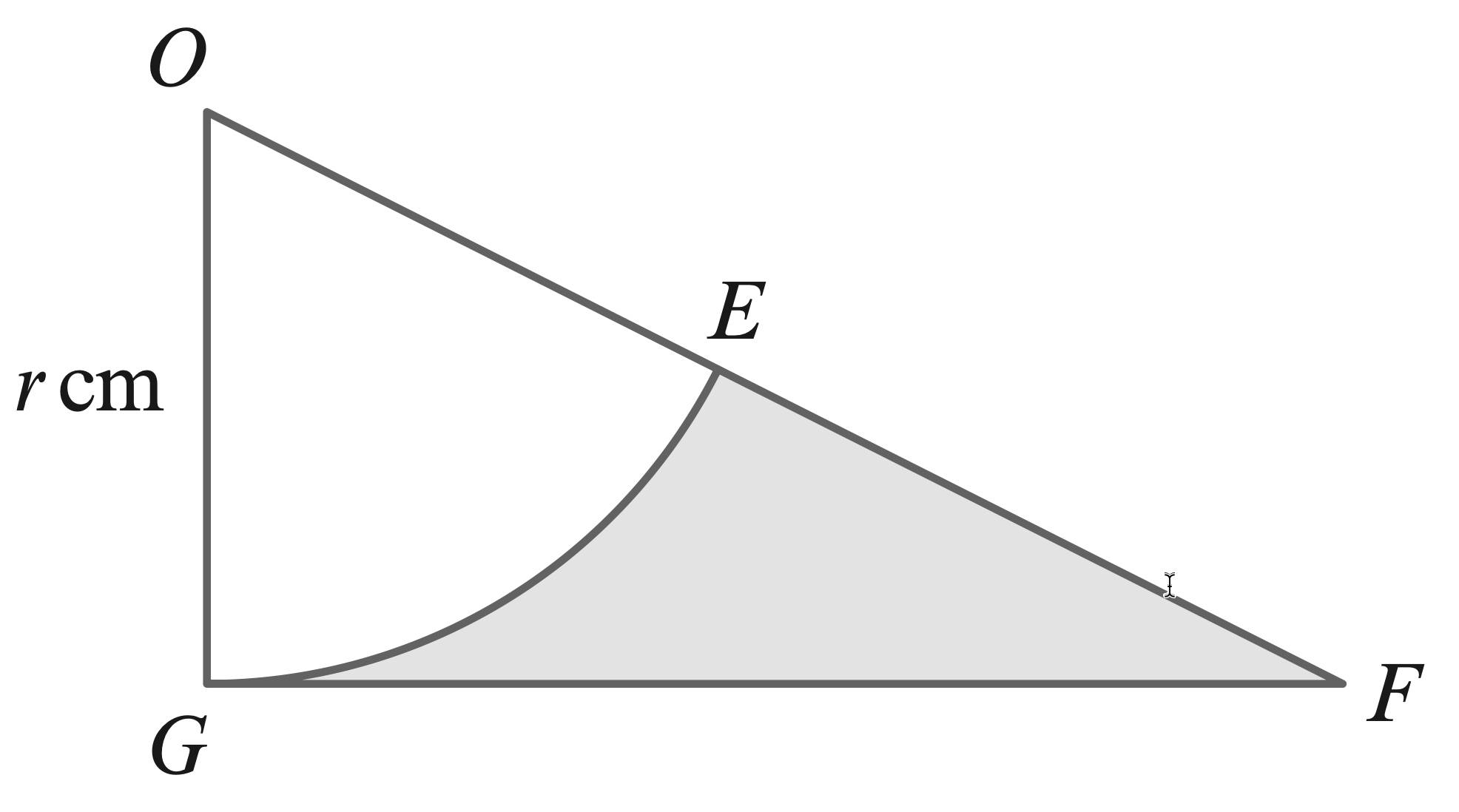21. The diagram shows a sector, $E O G$, of a circle, centre $O$, with radius $r \mathrm{~cm} .$ The line $G F$ is a tangent to the circle at $G$, and $E$ is the midpoint of $O F$.
(a) The perimeter of the shaded region is $P \mathrm{~cm}$. Show that $P=\dfrac{r}{3}(3+3 \sqrt{3}+\pi)$.
(b) The area of the shaded region is $A \mathrm{~cm}^{2}$. Show that $A=\dfrac{r^{2}}{6}(3 \sqrt{3}-\pi)$.

Since $E$ is the midpoint of of, $O E=E F=r \mathrm{~cm}\\\\$.
Since $GF$ a tangent, $OG\perp GF.\\\\$
$\therefore\ \triangle O G F \text{ is a } 30^{\circ}-60^{\circ} \text{ right triangle.}\\\\$
$\therefore\ G F=\sqrt{3} \mathrm{~cm}\\\\$
length of arc GF $=r \cdot \dfrac{\pi}{3} \mathrm{~cm}\\\\$
\begin{aligned} \therefore P &=r+\sqrt{3} r+r \cdot \dfrac{\pi}{3} \\\\ &=\dfrac{r}{3}(3+3 \sqrt{3}+\pi)\\\\ \end{aligned}
\begin{aligned} &\textbf{ area of sector OEG}\\\\ =&\ \dfrac{1}{2} r^{2}\left(\dfrac{\pi}{3}\right) \\\\ =&\ \dfrac{\pi}{6} r^{2}\\\\ &\textbf{area of shaded region}\\\\ =& \text { area of triangle - area of rector } \\\\ =&\ \dfrac{1}{2} r \cdot \sqrt{3} r-\dfrac{\pi}{6} r^{2} \\\\ =&\ \dfrac{\sqrt{3}}{2} r^{2}-\dfrac{\pi}{6} r^{2} \\\\ =&\ \dfrac{r^{2}}{6}(3 \sqrt{3}-\pi) \end{aligned}

22.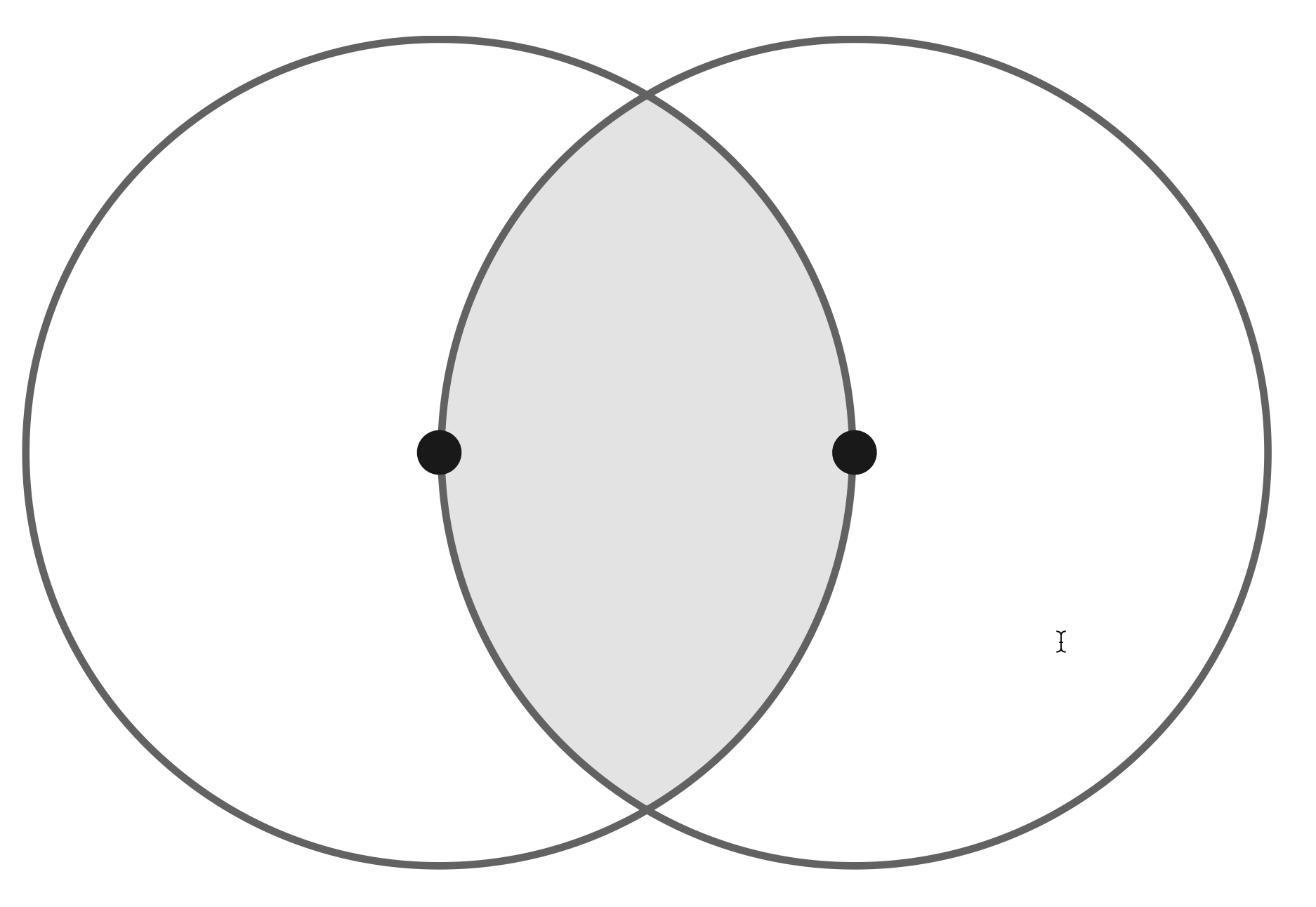23. The diagram shows two circles with radius $r \mathrm{~cm}$. The centre of each circle lies on the circumference of the other circle. Find, in terms of $r$, the exact area of the shaded region.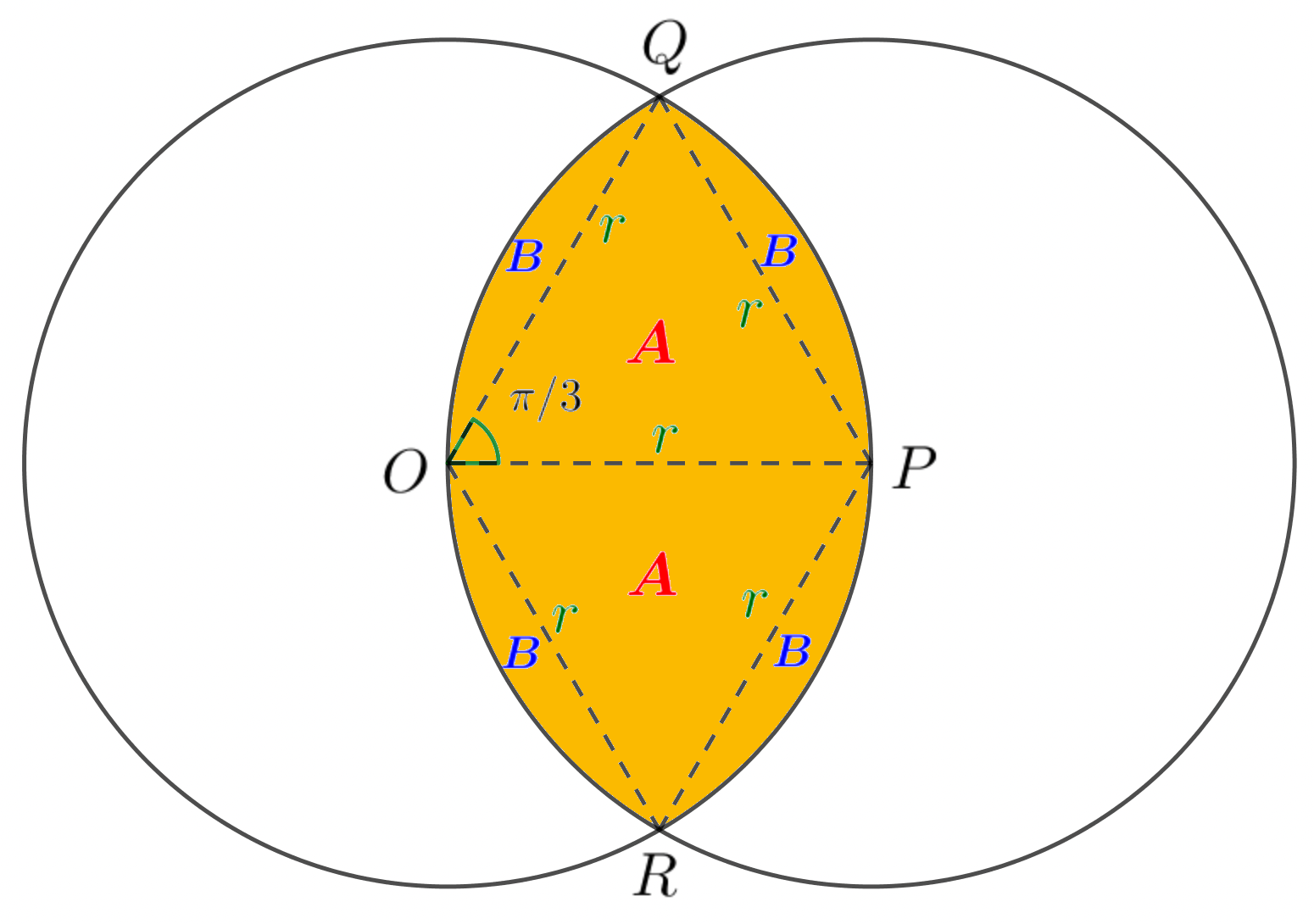Let $A$ and $B$ denote the area of respective regions.
$\therefore\ A=$ area of equilateral $\triangle\\\\$
$\quad\ =\dfrac{\sqrt{3}}{4} r^{2}\\\\$
\begin{aligned} A+B&= \text{ area of sector}\\\\ &=\dfrac{1}{2} r^{2}\left(\dfrac{\pi}{3}\right) \\\\ &=\dfrac{\pi}{6} r^{2} \\\\ B &=A+B-A \\\\ &=\dfrac{\pi}{6} r^{2}-\dfrac{\sqrt{3}}{4} r^{2} \\\\ &=\dfrac{r^{2}}{12}(2 \pi-3 \sqrt{3})\\\\ \textbf{Area of } & \textbf{ shaded region}\\\\ &=2 A+4 B \\\\ &=2(A+B)+2 B \\\\ &=2\left(\dfrac{\pi}{6} r^{2}\right)+\dfrac{r^{2}}{6}(2 \pi-3 \sqrt{3}) \\\\ &=\dfrac{r^{2}}{6}(4 \pi-3 \sqrt{3}) \end{aligned}

24.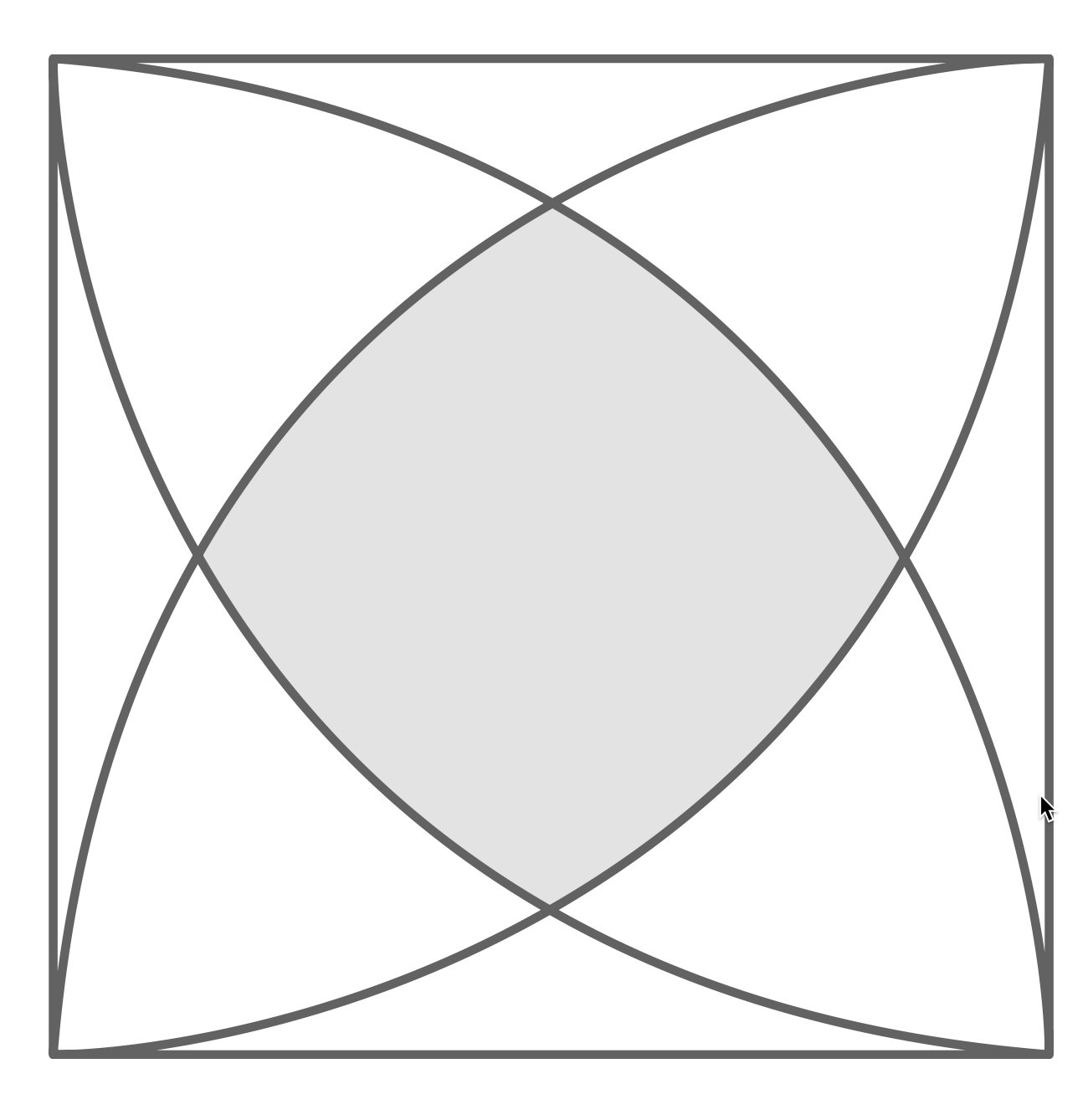25. The diagram shows a square of side length $10$ cm. A quarter circle, of radius $10$ cm, is drawn from each vertex of the square. Find the exact area of the shaded region.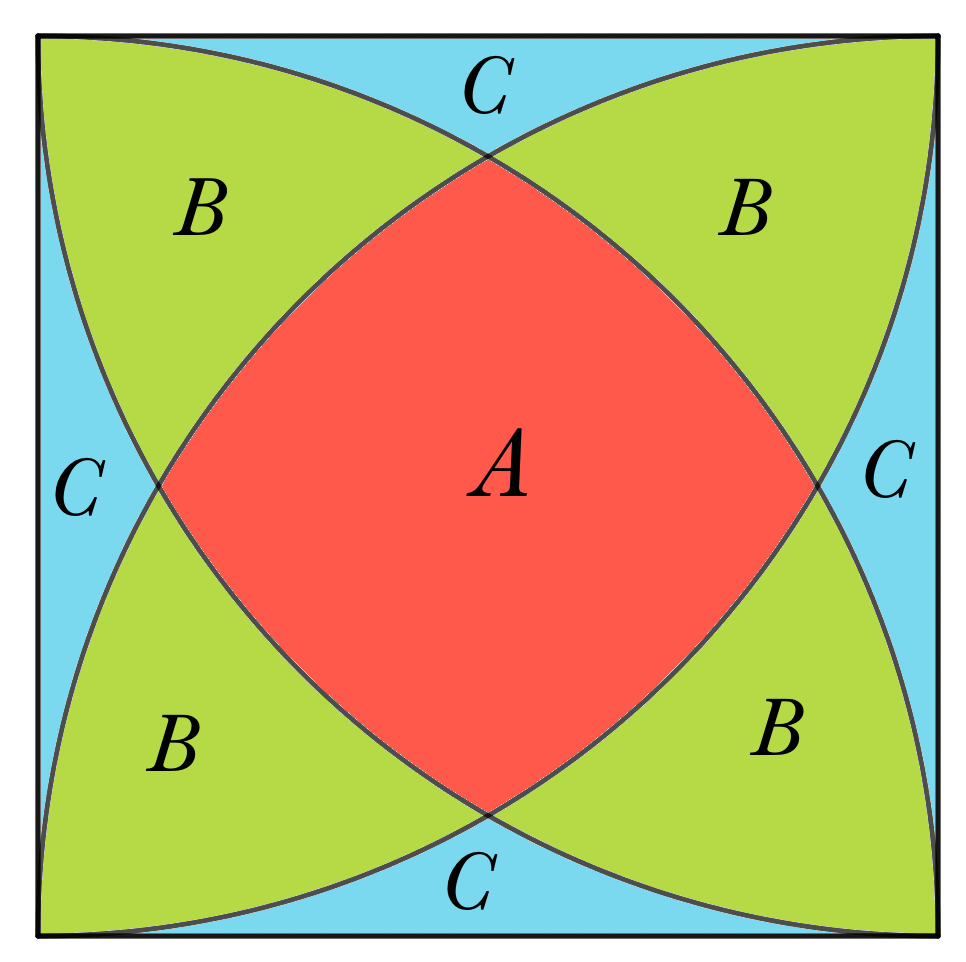Let $A, B$ and $C$ denot the areas of respective regions. Now, cosider the diagram as below.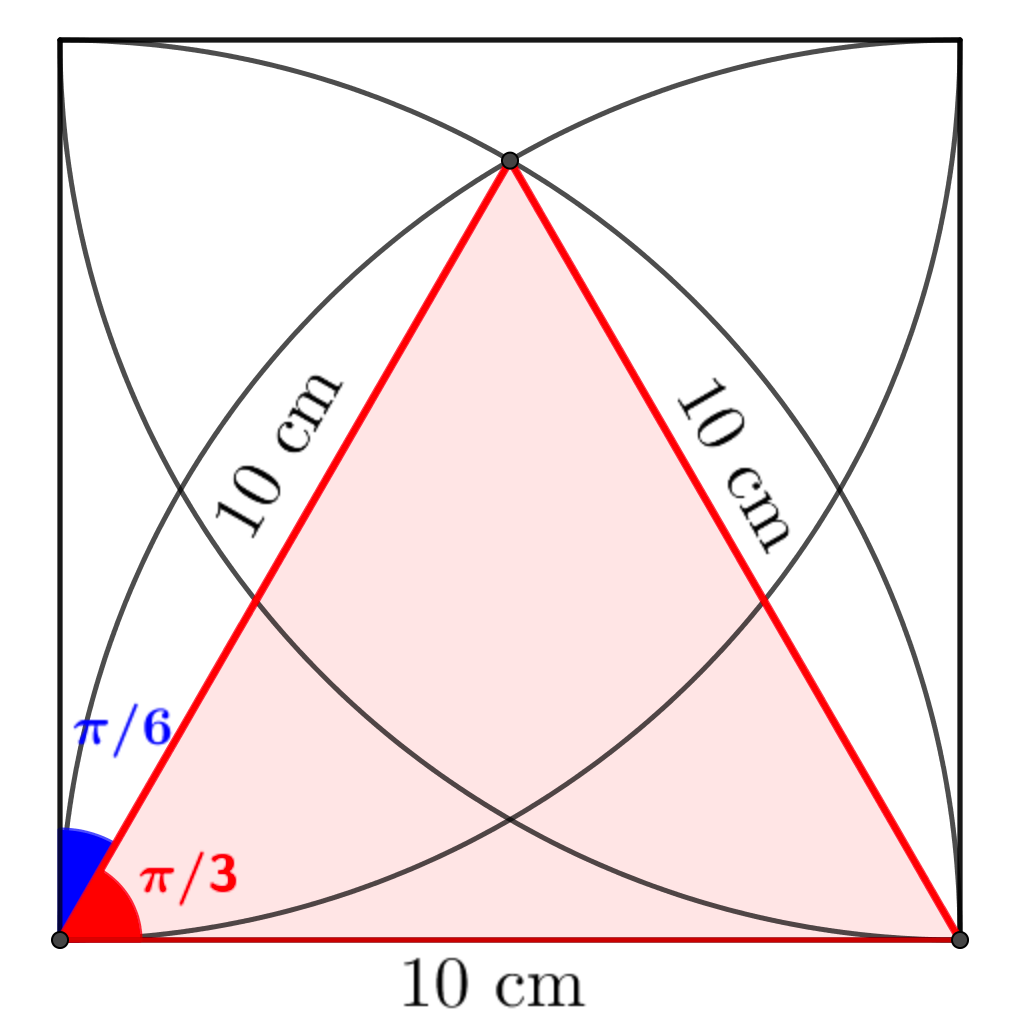$P Q=Q R=P R=$ radii of congruent circles.
$\therefore$ area of $\triangle P Q R=\dfrac{\sqrt{3}}{4}\left(10^{2}\right)=25 \sqrt{3} \mathrm{~cm}^{2}\\\\$
area of sector $P Q R=\dfrac{1}{2}\left(10^{2}\right) \dfrac{\pi}{3}\\\\$
$\hspace{3cm} =\dfrac{50 \pi}{3} \mathrm{~cm}^{2}\\\\$
Let $E$ be the area of yellow-shaded region as shown below.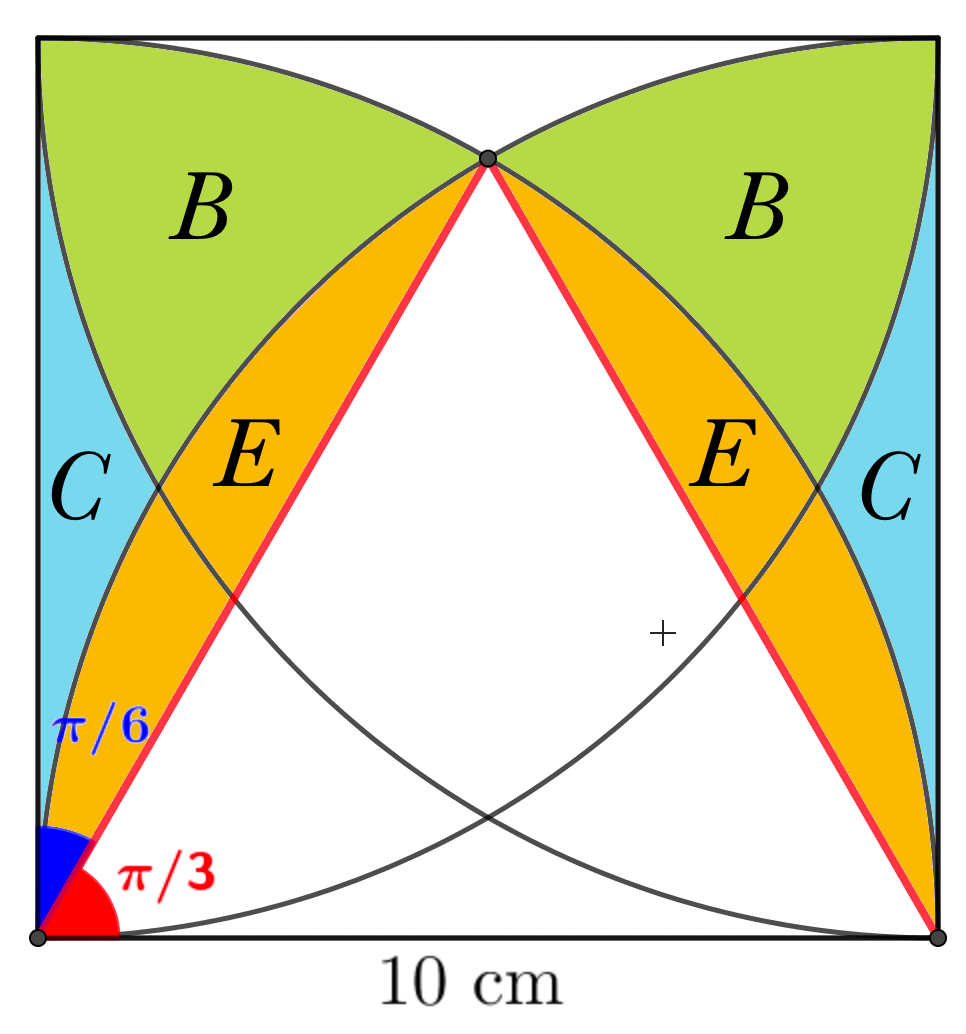\begin{aligned} \therefore\ E &=\text { area of sector }-\text { area of } \triangle \\\\ &=\dfrac{50 \pi}{3}-25 \sqrt{3} \mathrm{~cm}^{2} \\\\ B+C+E &=\dfrac{1}{2}\left(10^{2}\right)\left(\dfrac{\pi}{6}\right) \\\\ &=\dfrac{25 \pi}{3} \mathrm{~cm}^{2} \\\\ \therefore\ B+C &=\dfrac{25 \pi}{3}-\dfrac{50 \pi}{3}+25 \sqrt{3} \\\\ &=25 \sqrt{3}-\dfrac{25 \pi}{3} \mathrm{~cm}^{2}\\\\ \end{aligned}
Now recall to give diagram.\begin{aligned} A &=\text { area of square }-4(B+C) \\\\ &=10^{2}-4\left[25 \sqrt{3}-\dfrac{25 \pi}{3}\right] \\\\ &=100-100\left[\sqrt{3}-\dfrac{\pi}{3}\right] \\\\ &=100\left[1-\sqrt{3}+\dfrac{\pi}{3}\right] \mathrm{cm}^{2} \end{aligned}

26.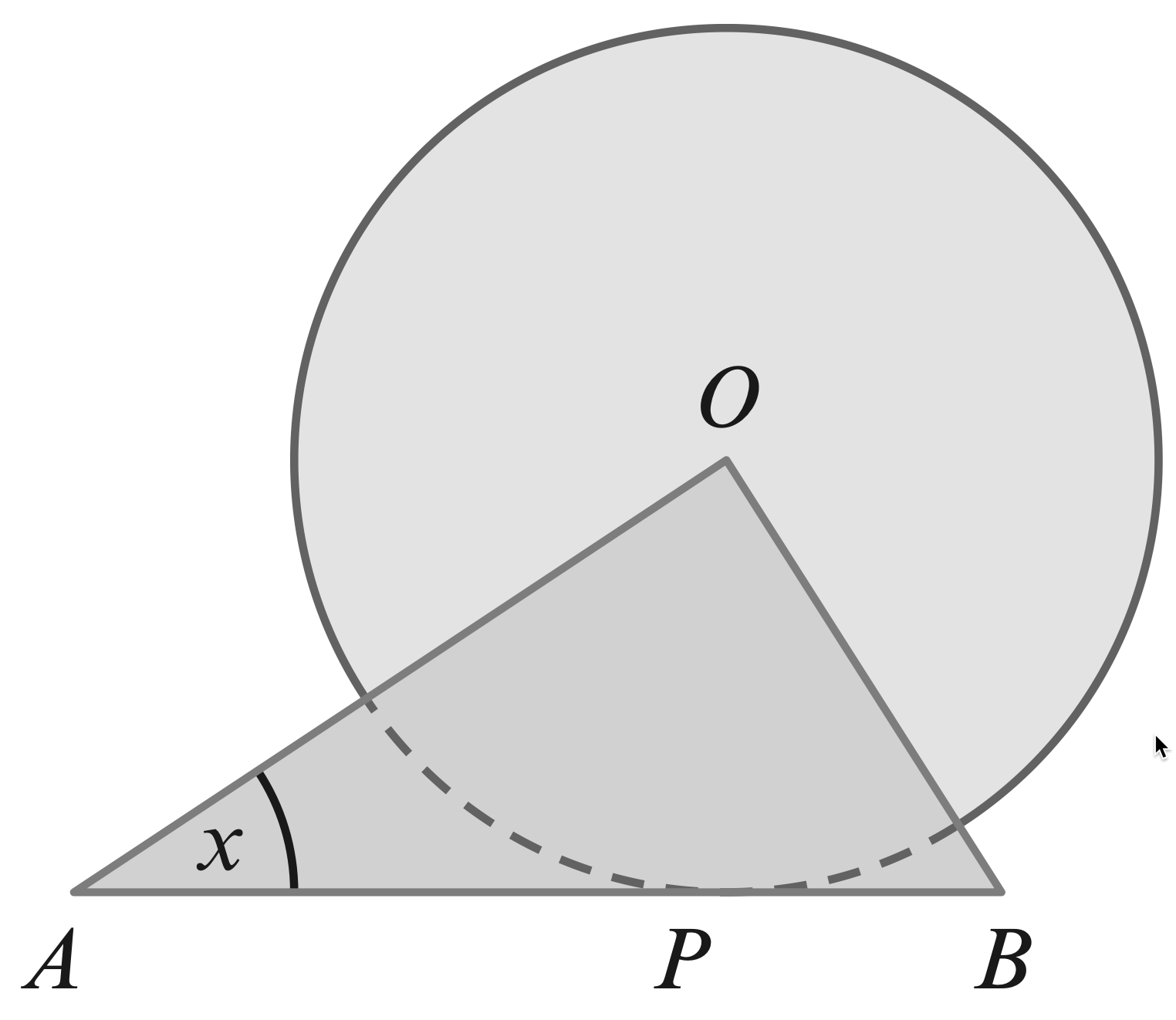27. The diagram shows a circle with radius $1$ cm, centre $O$. Triangle AOB is right angled and its hypotenuse AB is a tangent to the circle at $P$. Angle $BAO = x$ radians.
(a) Find an expression for the length of AB in terms of $\tan x$.
(b) Find the value of $x$ for which the two shaded areas are equal.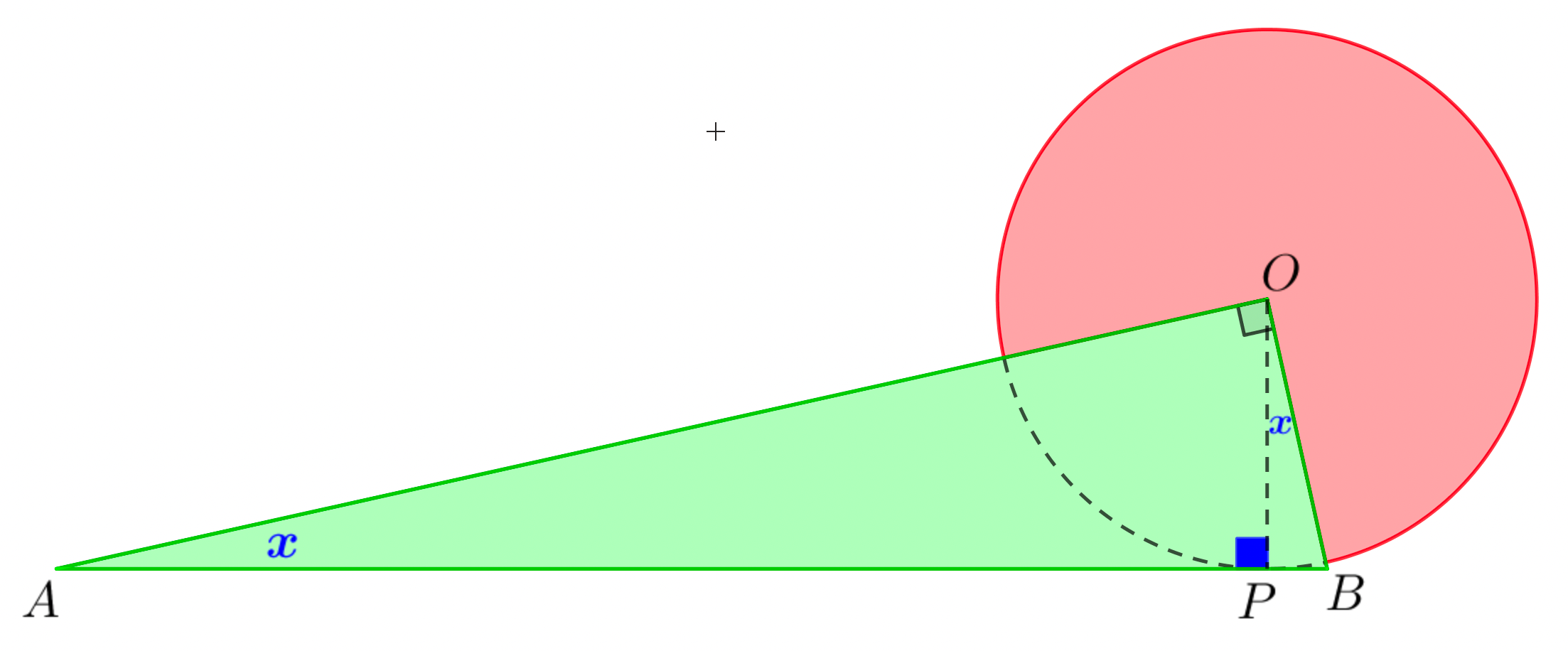\begin{aligned} \dfrac{1}{A P} &=\tan x \\\\ A P &=\dfrac{1}{\tan x} \\\\ \dfrac{P B}{1} &=\tan x \\\\ P B &=\tan x \\\\ A B &=A P+P B \\\\ &=\tan x+\dfrac{1}{\tan x}\\\\ \textbf{Area } & \textbf{ of triangle}\\\\ &=\dfrac{1}{2} \times A B \times O P \\\\ &=\dfrac{1}{2}\left(\tan x+\dfrac{1}{\tan x}\right)\\\\ \textbf{Area } & \textbf{ of sector}\\\\ &=\dfrac{1}{2}\left(\dfrac{3 \pi}{2}\right) \\\\ &=\dfrac{3 \pi}{4}\\\\ \textbf{By the problem}&\\\\ \dfrac{1}{2}\left(\tan x+\dfrac{1}{\tan x}\right) &=\dfrac{3 \pi}{4} \\\\ \dfrac{1}{2}\left(\dfrac{\sin x}{\cos x}+\dfrac{\cos x}{\sin x}\right) &=\dfrac{3 \pi}{4} \\\\ \dfrac{\sin ^{2} x+\cos ^{2} x}{2 \sin x \cos x} &=\dfrac{3 \pi}{4} \\\\ \dfrac{1}{\sin 2 x} &=\dfrac{3 \pi}{4} \\\\ \sin 2 x &=\dfrac{4}{3 \pi} \end{aligned}

28.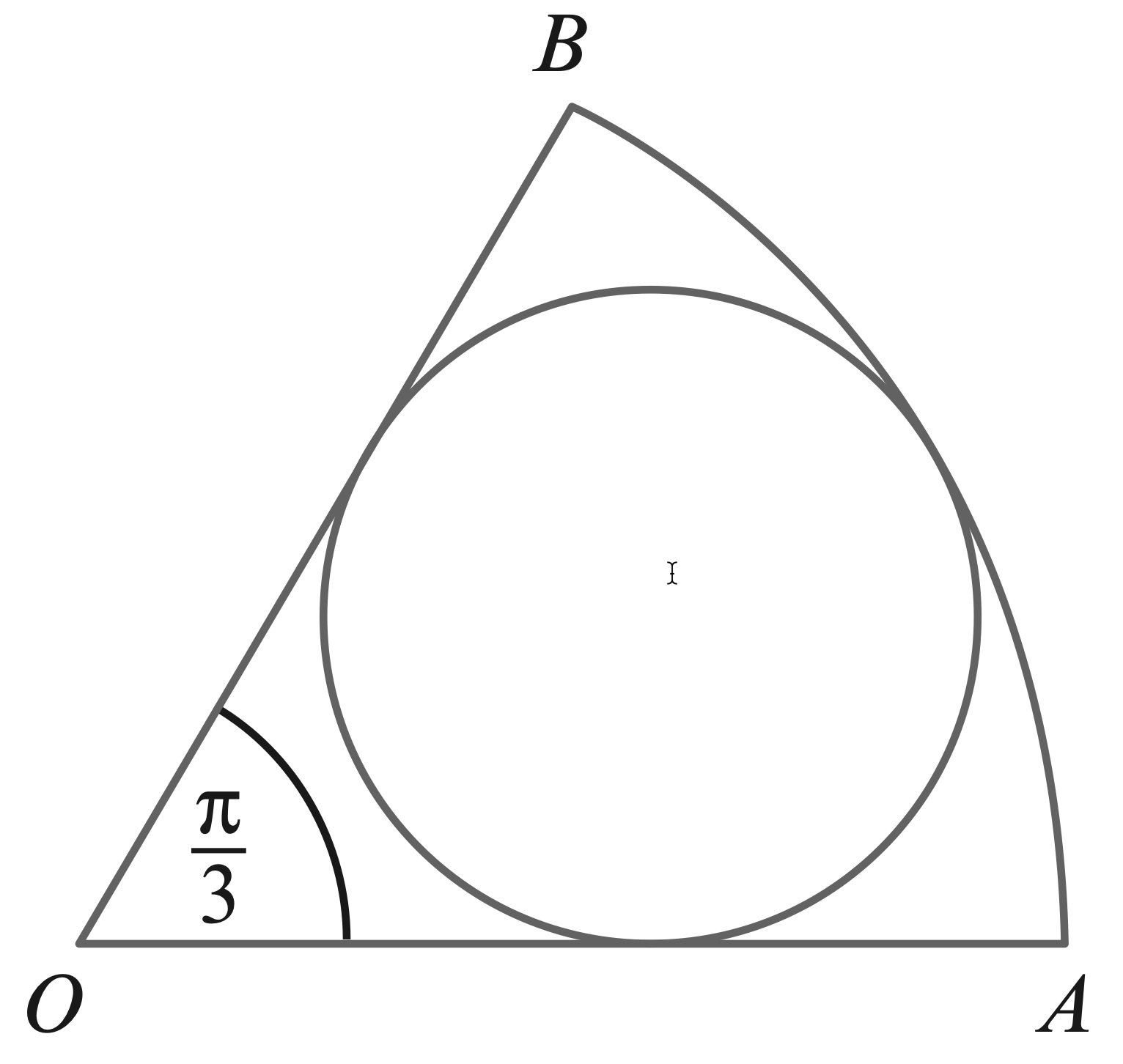29. The diagram shows a sector, $AOB$, of a circle, centre $O$, with radius $R$ cm and sector angle $\dfrac{π}{3}$ radians.
An inner circle of radius $r$ cm touches the three sides of the sector.
(a) Show that $R = 3r$.
(b) Show that $\dfrac{\text{area of inner circle}}{\text{area of sector}}=\dfrac{2}{3}$ .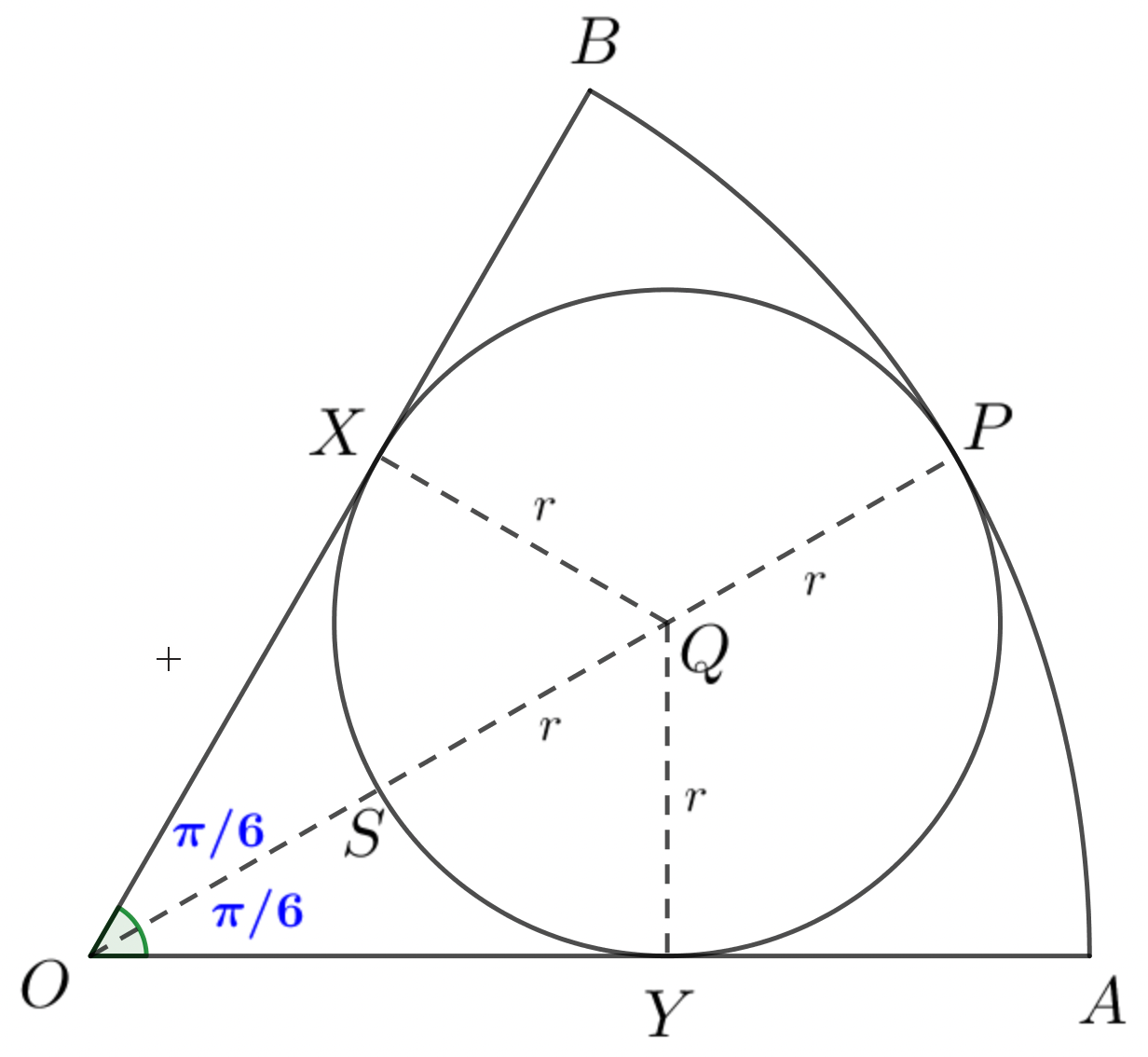let the $X, P, Y$ be the point of tangency as shown in figure.
The line joining the point $O$ and $P$ passes theough the centre of inscribed cirle.
Let that centre be $Q.\\\\$
$\therefore Q X=Q Y=QS=r\\\\$
Since $OP$ bisects $\angle AOB,\\\\$
$\angle A O P=\angle P O B=\dfrac{\pi}{6} \mathrm{rad}\\\\$
$\therefore \triangle OQX$ and $OQY$ are $30^{\circ}-60^{\circ}$ right triangle.
Thus, $OQ =2 r$ and $O P=R=3 r\\\\$.
\begin{aligned} \dfrac{\text { Area of inner circle }}{\text { Area of sector }}&=\dfrac{\pi r^{2}}{\dfrac{1}{2} R^{2}\left(\dfrac{\pi}{3}\right)}\\\\ &=\dfrac{\pi r^{2}}{\dfrac{1}{2} \cdot \dfrac{\pi}{3} \cdot 9 r^{2}} \\\\ &=\dfrac{2}{3} \end{aligned}

30.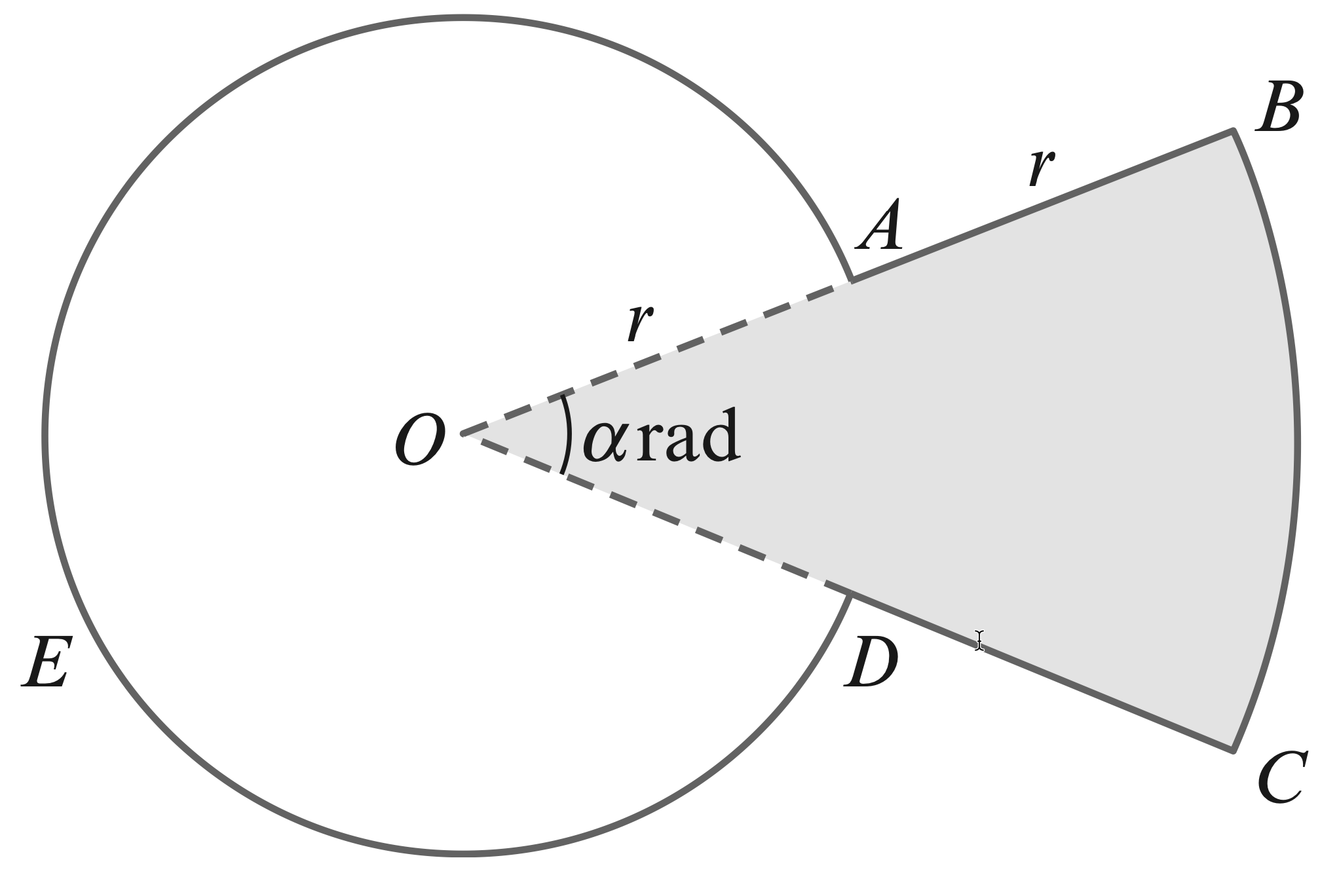31. The diagram shows a metal plate made by fixing together two pieces, $OABCD$ (shaded) and $OAED$ (unshaded). The piece $OABCD$ is a minor sector of a circle with centre $O$and radius $2r$. The piece $OAED$ is a major sector of a circle with centre $O$ and radius $r$. Angle $AOD$ is $\alpha$ radians. Simplifying your answers where possible, find, in terms of $\alpha, \pi$ and $r$,
(a) the perimeter of the metal plate,
(b) the area of the metal plate.
It is now given that the shaded and unshaded pieces are equal in area.
(c) Find $\alpha$ in terms of $\pi$.

\begin{aligned} l_{1} &=\text { length of arc AED. } \\\\ &=r(2 \pi-\alpha) \\\\ l_{2} &=\text { length of arc BD } \\\\ &=2 r \alpha\\\\ \textbf{Perimeter } & \textbf{ of metal plate}\\\\ &=l_{1}+l_{2}+2 r \\\\ &=r(2 \pi-\alpha)+2 r \alpha+2 r \\\\ &=r(2 \pi-\alpha+2 \alpha+2) \\\\ &=r(2 \pi+\alpha+2)\\\\ \textbf{By the problem,}&\\\\ \text { shaded are } &=\text { unshaded area } \\\\ \dfrac{1}{2}(2 r)^{2} \alpha &=\dfrac{1}{2} r^{2}(2 \pi-\alpha) \\\\ 4 \alpha &=2 \pi-\alpha \\\\ 5 \alpha &=2 \pi \\\\ \alpha &=\dfrac{2 \pi}{5} \end{aligned}

စာဖတ်သူ၏ အမြင်ကို လေးစားစွာစောင့်မျှော်လျက်!